## Séminaire System X, 6 Juin 2018

Etienne Côme COSYS/GRETTIA/IFSTTAR

# Generative models

• Describe the data generation process (prediction)
• May include latent variables (clusters or latent space)
• Helpfull to get a synthetic view of the system
• May be use to generate synthetic population / links with simulations

# Visualize and analyze Vélib' data

## Stocks

in open-data (Real-Time Apps)

## Flows

Origines / Destinations sometime in open-data (London, New-York, Boston,...) frequently not (France)

# Animated bikes stocks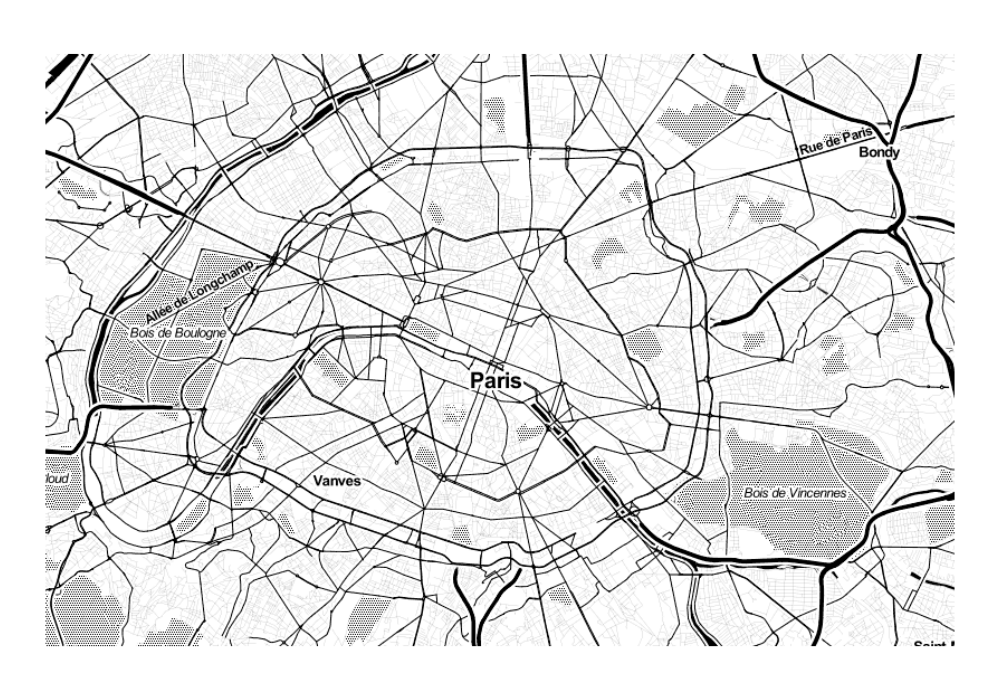# Animated bikes stocks

• A problem of balance !
• A regulation challenge
• A move // a repetitive pulse

# Stocks data : vlsstat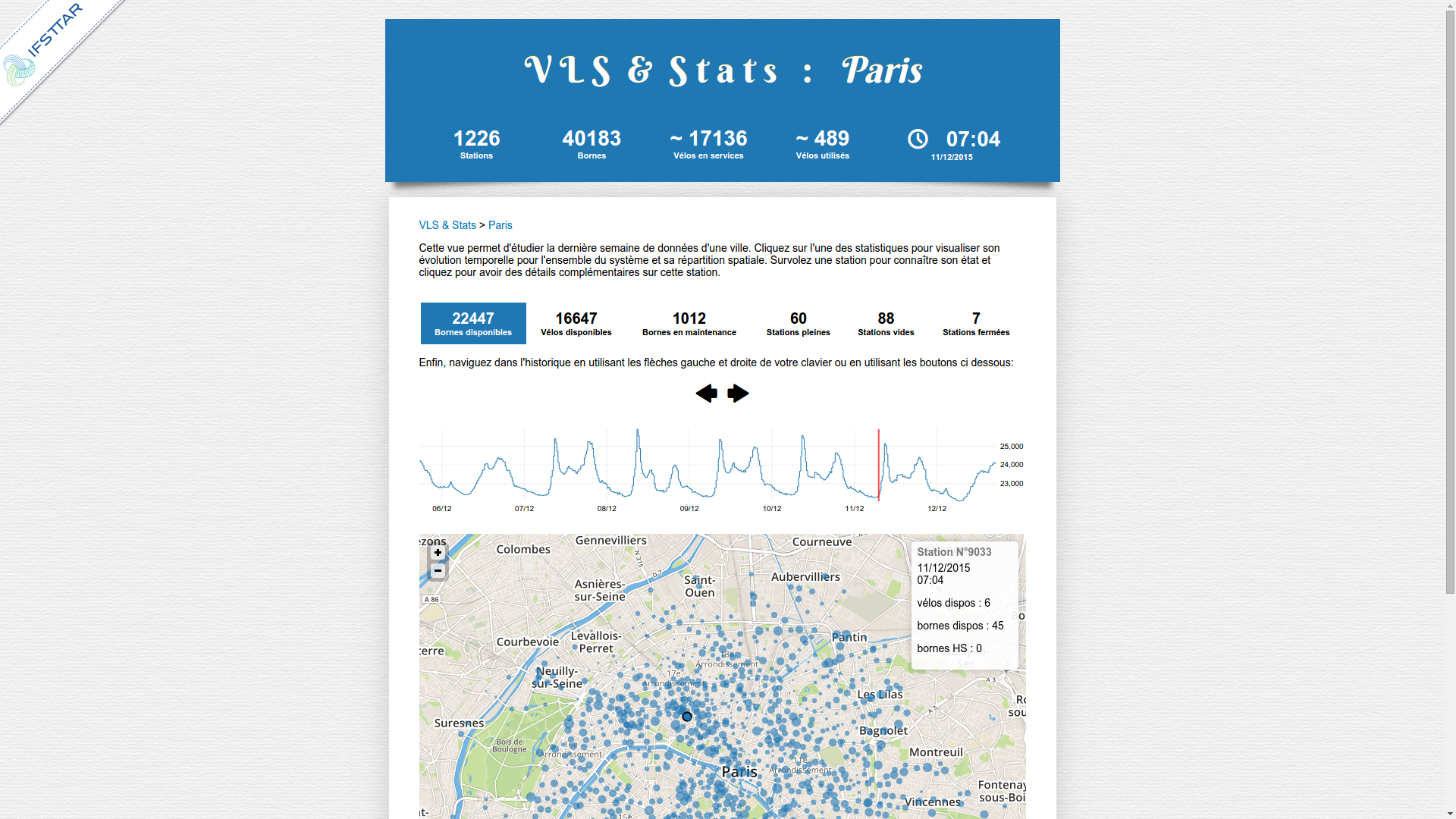# Stocks data : vlsstat

### Functional data clustering on stocks series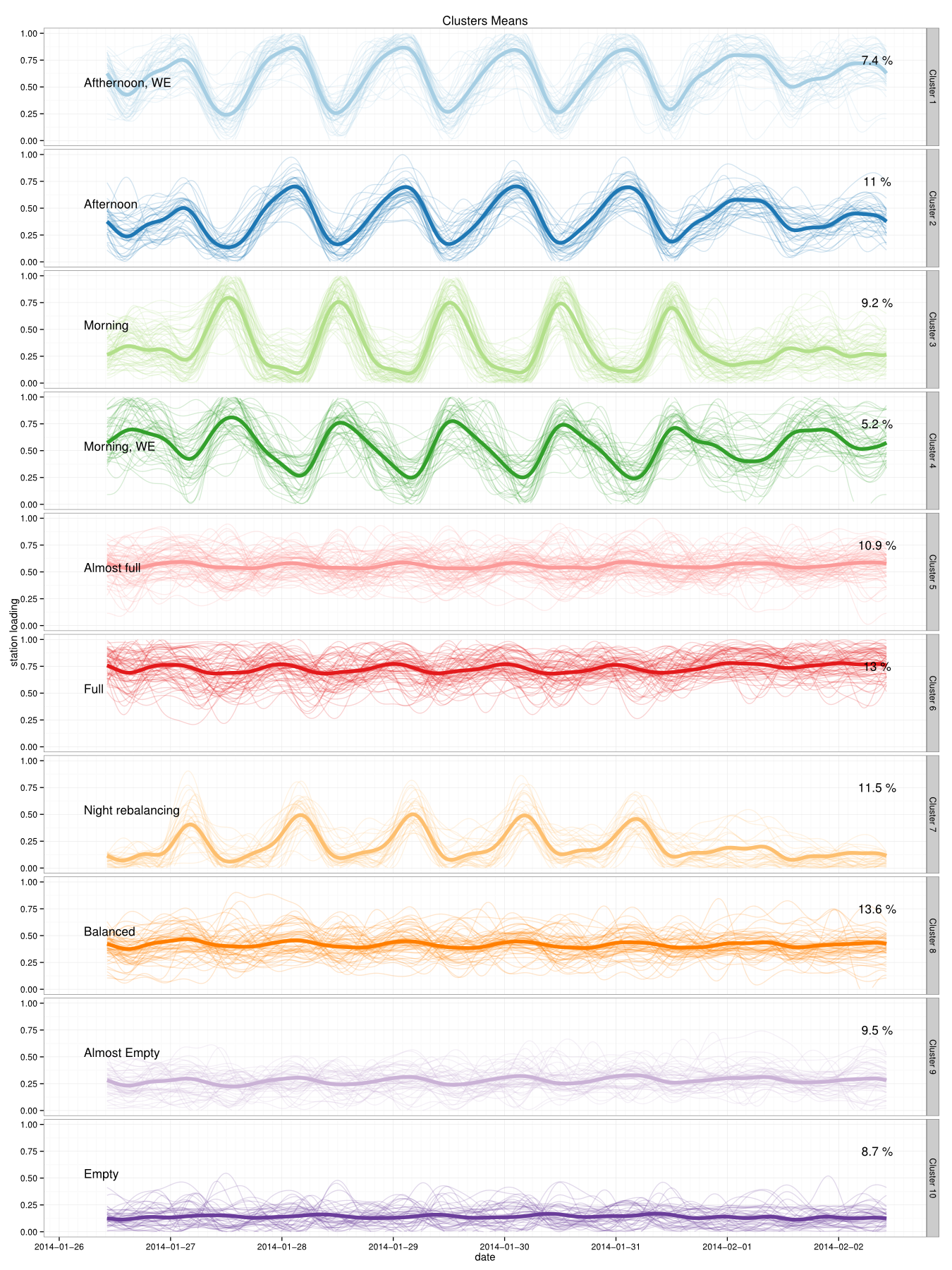The Discriminative Functional Mixture Model for the Analysis of Bike Sharing Systems [preprint]

### Functional data clustering on stocks series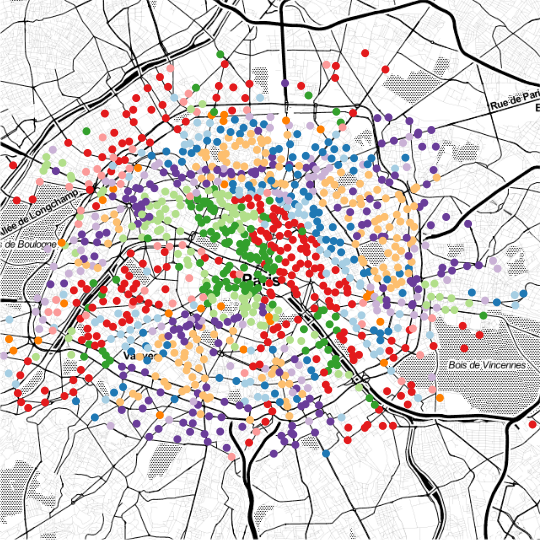The Discriminative Functional Mixture Model for the Analysis of Bike Sharing Systems [preprint]

### Functional data clustering on stocks series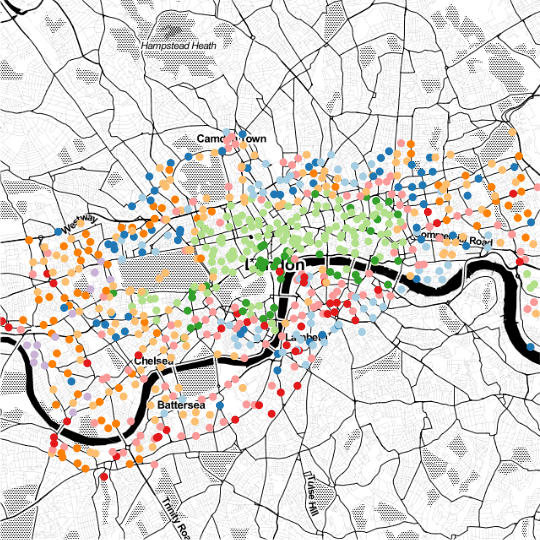The Discriminative Functional Mixture Model for the Analysis of Bike Sharing Systems [preprint]

### Functional data clustering on stocks series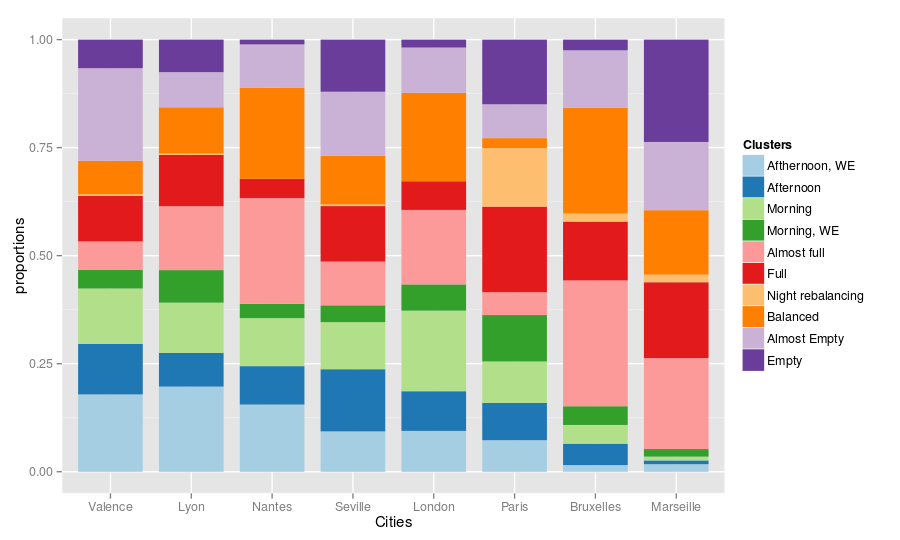The Discriminative Functional Mixture Model for the Analysis of Bike Sharing Systems [preprint]

# Station clustering model

• $X_{sdt}$ (observed): number of bikes comming/leaving
• $Z_s$ (latent): station s cluster
• $W_d$ (observed): (week / week end), meteo, ...

## Simple generative model :

$$Z_s\sim\mathcal{Multinomial}(1,\pi)$$ $$X_{sdt}|\{Z_{sk}=1,W_{dl}=1\}\sim\mathcal{Poisson}(\alpha_s\lambda_{klt})$$ + constraints $\sum_{l,t}D_l\lambda_{klt}=DT, \forall k \in\{1,...,K\}$,
with $D_l$ number of days in $l$.

# Station clustering model

• independent poissons distristributions
• stations scaling factors $\alpha_s$
• categorical information on days (day of week, meteo,...)

## Completed - Likelihood :

$$Lc(\mathbf{\Theta};\mathbf{X},\mathbf{Z},\mathbf{\alpha},\mathbf{W})=\sum_{s,k}Z_{sk}\log\left(\pi_{k}\prod_{d,t,l}po(X_{sdt};\alpha_s\lambda_{klt})^{W_{dl}}\right)$$ Estimation by EM

# Urban dynamics through the observed flows (2 month of O/D data in Paris)

#### http://www.comeetie.fr/galerie/velib/# Urban dynamics through the observed flows

• An interesting viewpoint
• Easy interpretation
• Crossing with contextual data
• ...

### Crossing with contextual data // socio-eco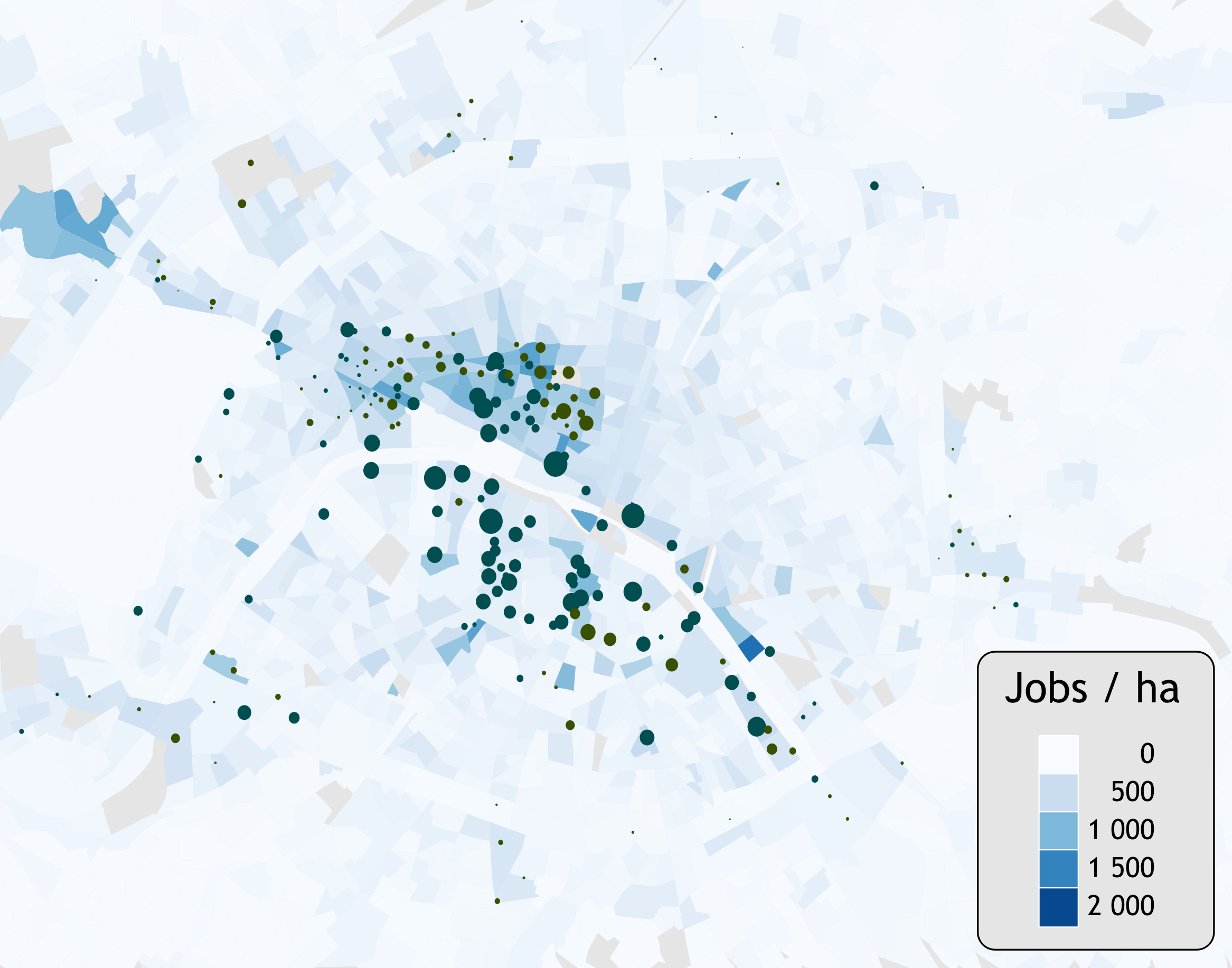### Crossing with contextual data // socio-eco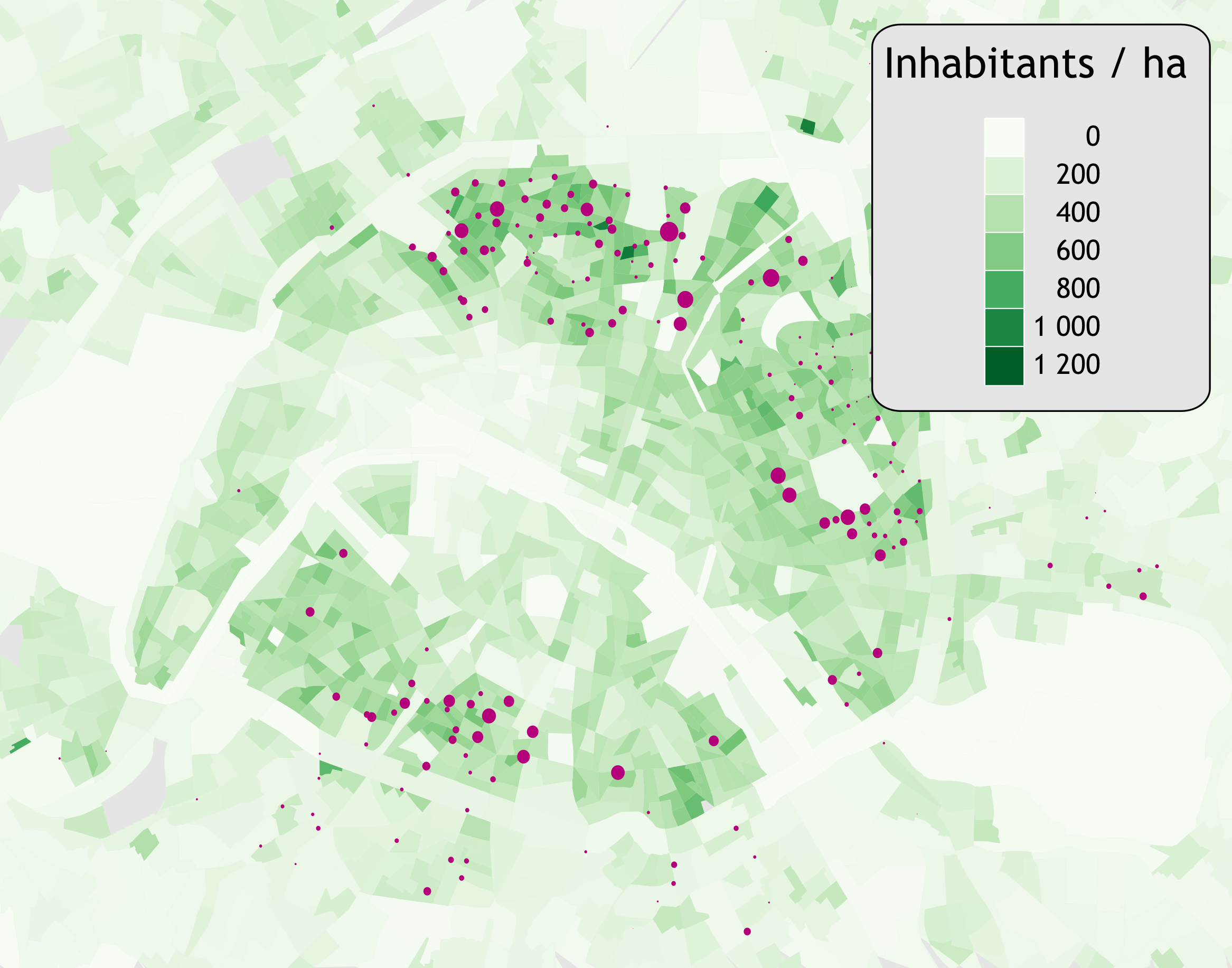### Crossing with contextual data // socio-eco

 hab/ha emp/ha serv/ha com/ha * 162 237 4.2 3.7 Leisure (1) 367 189 6.3 4.4 Leisure (2) 261 322 7.7 6.9 Parks 172 90 2 1.7 Stations 209 206 2.4 1.8 Mixed 375 108 3.8 2.7 Jobs(1) 138 409 4.5 2.8 Jobs(2) 157 456 5.7 5.6 Average 301 163 3.8 2.8

# Latent Dirichlet Allocation

#### For dynamic Origine-Destination matrices analysisLocal stationarity of BSS behaviour / OD
Small bags of successive trips $\approx$ stationarity of OD
Documents (bags of words) = bags of successive trips (5000)
With :
• Words = Origin/Destination couples
• Topics = Latent activities

# Latent Dirichlet Allocation

#### For dynamic Origine-Destination matrices analysis

For each latent activity $a$, draw its template : $\Lambda_a\sim\mathcal{Dirichlet}(\beta)$
For each bag of trips
Draw the proportion of activities : $\pi_t \sim \mathcal{Dirichlet}(\alpha)$
For each trips
Draw its activity
$A \sim \mathcal{Multinomial}(1,\pi_t)$
Draw an OD using the activity template
$D \sim \mathcal{Multinomial}(1,\Lambda_A)$

# OD decomposition resultsModel selection with perplexity analysis
(clear drop for K=5)

# Latent activity template analysis

#### Expected stations balances

Draw $N_{tr}$, (number of trips) using $\Lambda_a$ : $$OD\sim\mathcal{Multinomial}(N_{tr},\Lambda_a)$$ Compute the balance (incoming bikes - leaving bikes) for a station $s$ : $$B_s=\sum_jOD_{js}-\sum_jOD_{sj}$$ Compute the expectation for each station $\mathbb{E}[\mathbf{B}]=N_{tr}(\Lambda_a^t-\Lambda_a)\mathbf{1}$

# Stations Balances : home→work# Stations Balances: work→home# Stations Balances : evening# Gravity-LDA

#### LDA extension for taking stations context into-accounts

Replace the O/D matrix templates $\Lambda_k$ by a parametric form which depends on :
• context of departure station, $\mathbf{x_u}$
• context of arrival station, $\mathbf{x_v}$
• distance between $u$ and $v$, $\mathbf{x_{uv}^{da}}$
\begin{equation} \Lambda_{\,uv}(\Theta_k) \,=\, \frac{ \exp(\mathbf{\theta_{k}^{d}}^\top \mathbf{x_{u}} + \mathbf{\theta_{k}^{a}}^\top \mathbf{x_{v}} + \mathbf{\theta_{k}^{da}}^\top \mathbf{x_{uv}^{da}}) } {\sum\limits _{u,v}\; \exp(\mathbf{\theta_{k}^{d}}^\top \mathbf{x_{u}} + \mathbf{\theta_{k}^{a}}^\top \mathbf{x_{v}} + \mathbf{\theta_{k}^{da}}^\top \mathbf{x_{uv}^{da}}) } \end{equation}

# Gravity-LDA

#### LDA extension for taking stations context into-accounts

Replace the O/D matrix templates $\Lambda_k$ by a parametric form which depends on :
• context of departure station, $\mathbf{x_u}$
• context of arrival station, $\mathbf{x_v}$
• distance between $u$ and $v$, $\mathbf{x_{uv}^{da}}$
\begin{equation} \Lambda_{\,uv}(\Theta_k) \,=\, \frac{ \exp(\mathbf{\theta_{k}^{d}}^\top \mathbf{x_{u}} + \mathbf{\theta_{k}^{a}}^\top \mathbf{x_{v}} + \mathbf{\theta_{k}^{da}}^\top \mathbf{x_{uv}^{da}}) } {\sum\limits _{u,v}\; \exp(\mathbf{\theta_{k}^{d}}^\top \mathbf{x_{u}} + \mathbf{\theta_{k}^{a}}^\top \mathbf{x_{v}} + \mathbf{\theta_{k}^{da}}^\top \mathbf{x_{uv}^{da}}) } \end{equation}

# Conclusion on OD analysis

#### Results

• Easy segmentation of time
• Compact model of the system few template
• Evidences of clear cycles
• Also used for other type of data Bluetooth, smartcard,....
• Can be interesting for simulation
• Only satified demand is taken into account (users that do not find bikes!)

#### Extension

• Socio-economic factors + od factors (distances, type of roads) can be integrated
$\Rightarrow$ Usefull for new station planning (system extension)

# Bonus : cities portraits at Night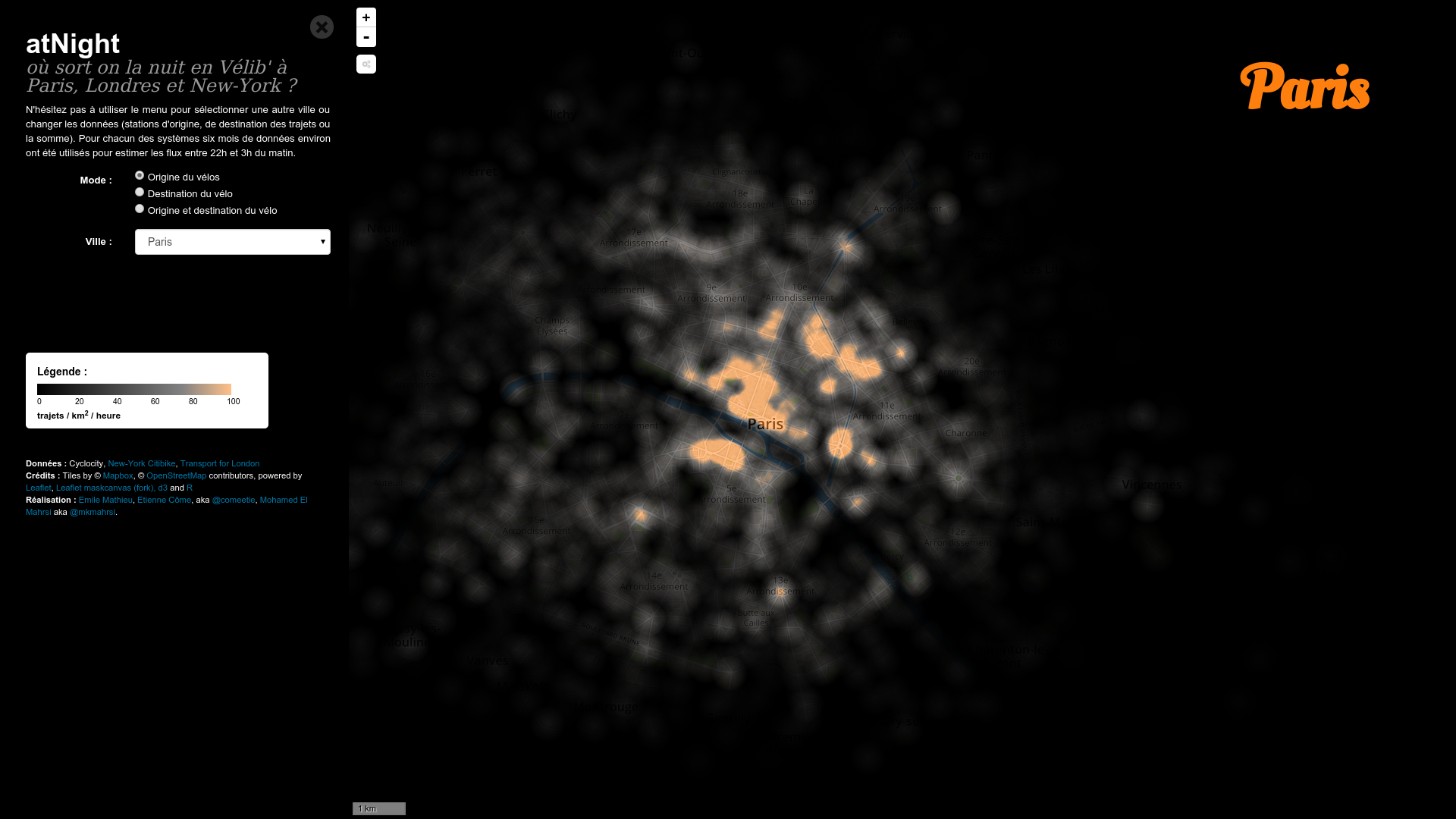# Bonus : cities portraits : atNight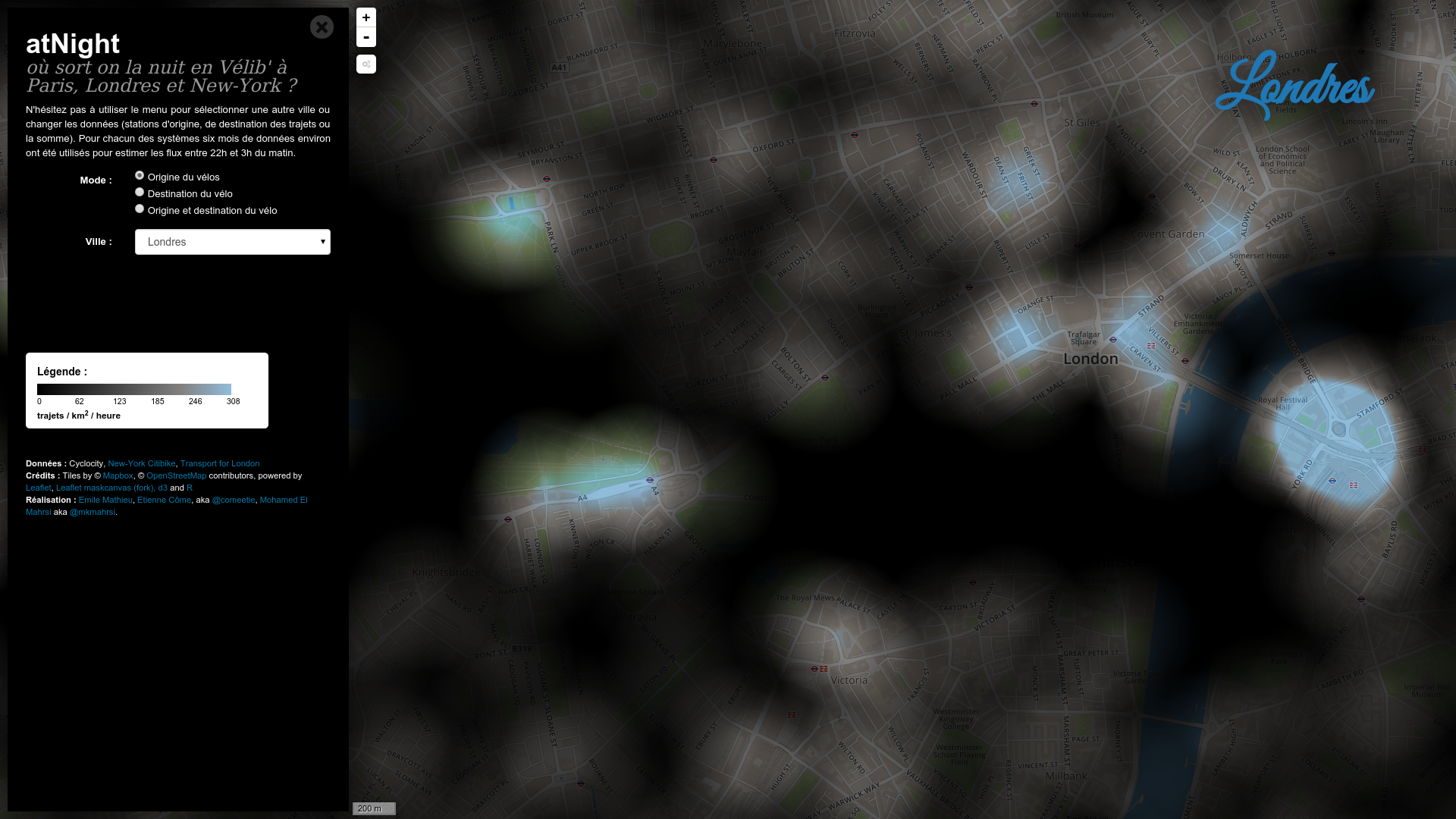# Transit networks analysis# Smart-card data form

• anonymous user id (changed every 3 month)
• type of card
• stop id (+line and direction for the bus)
• date and time

#### Open-Data ? (ex: "Ile de France Mobilité" in aggregated form)# Smart-card data form

• anonymous user id (changed every 3 month)
• type of card
• stop id (+line and direction for the bus)
• date and time

#### A particular field : user id# A massive dataset

• anonymous user id (changed every 3 month)
• type of card
• stop id (+line and direction for the bus)
• date and time

#### What to do without user ids ?

• Analyzing in-flow volumes
• Days clustering
→ outliers detection, mid term prediction,...
• Stations clustering
→ spatial analysis of the demand, ...

#### What to do with user ids ?

• Destination inference
→ transfers analysis, dynamical OD matrices,...
• Short term OD matrix prediction
• Users clustering

# Analyzing in-flow volumes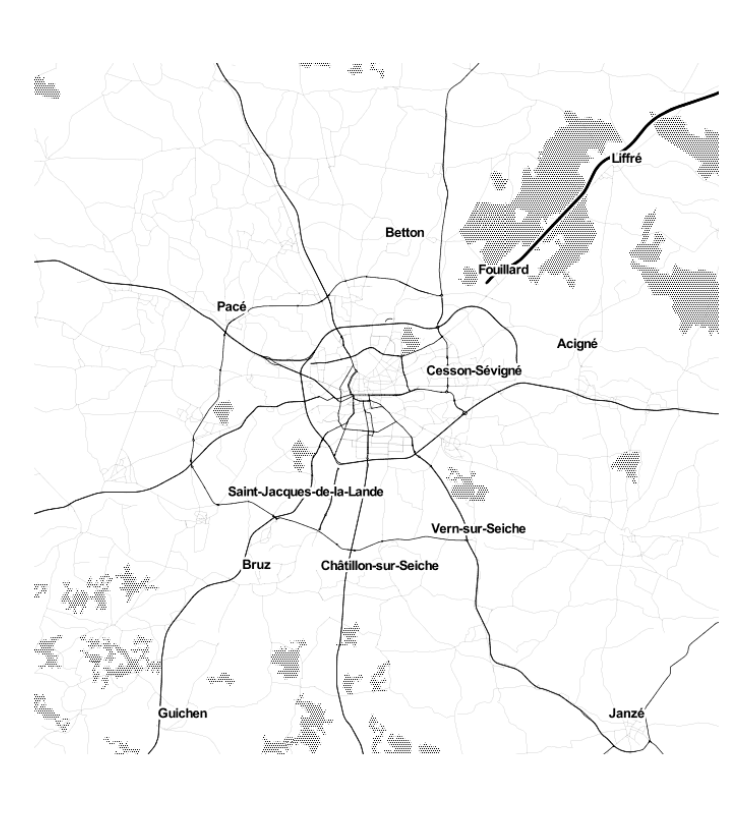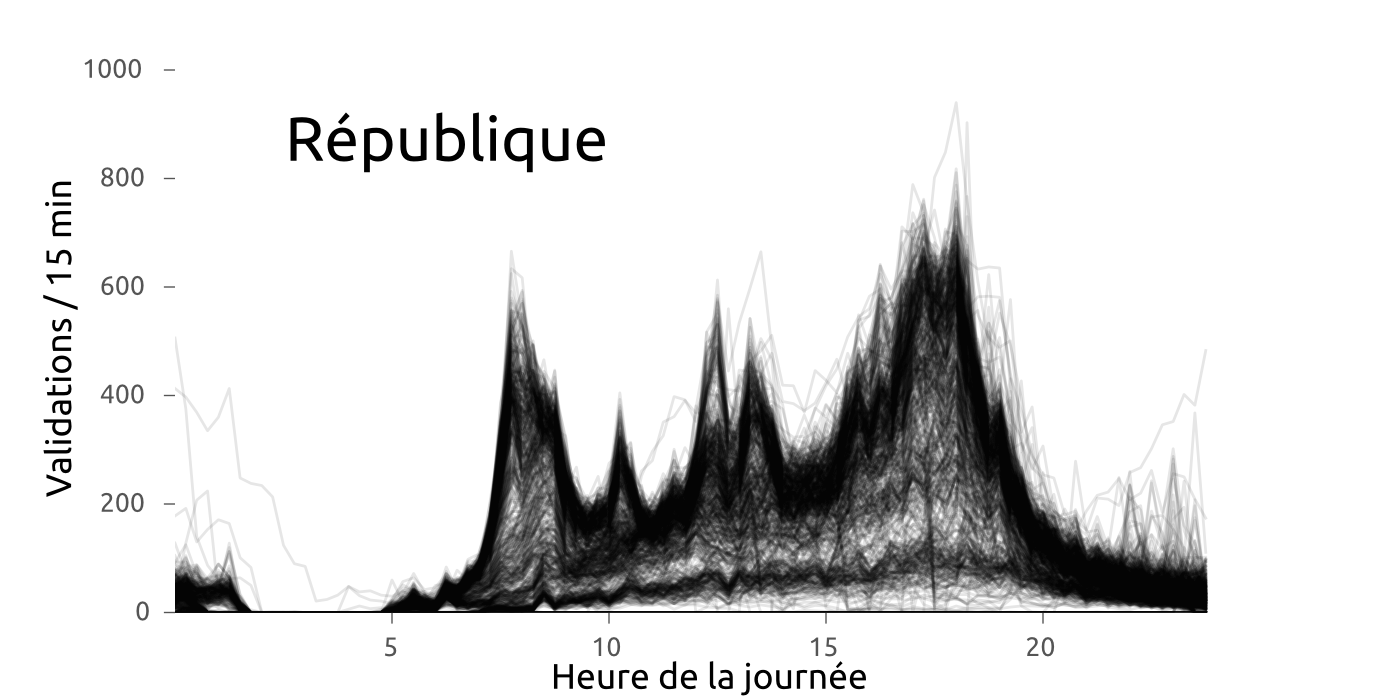#### Profiles of the demand with spatio-temporal variations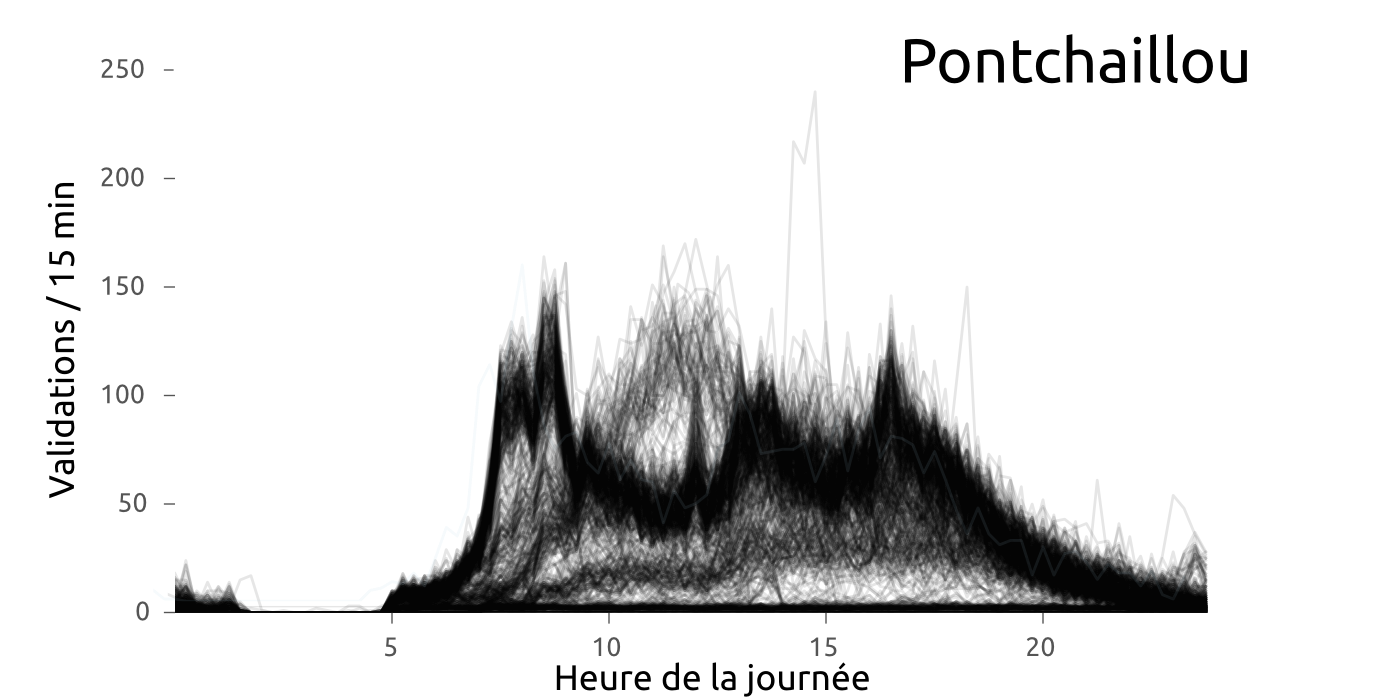#### Profiles of the demand with spatio-temporal variations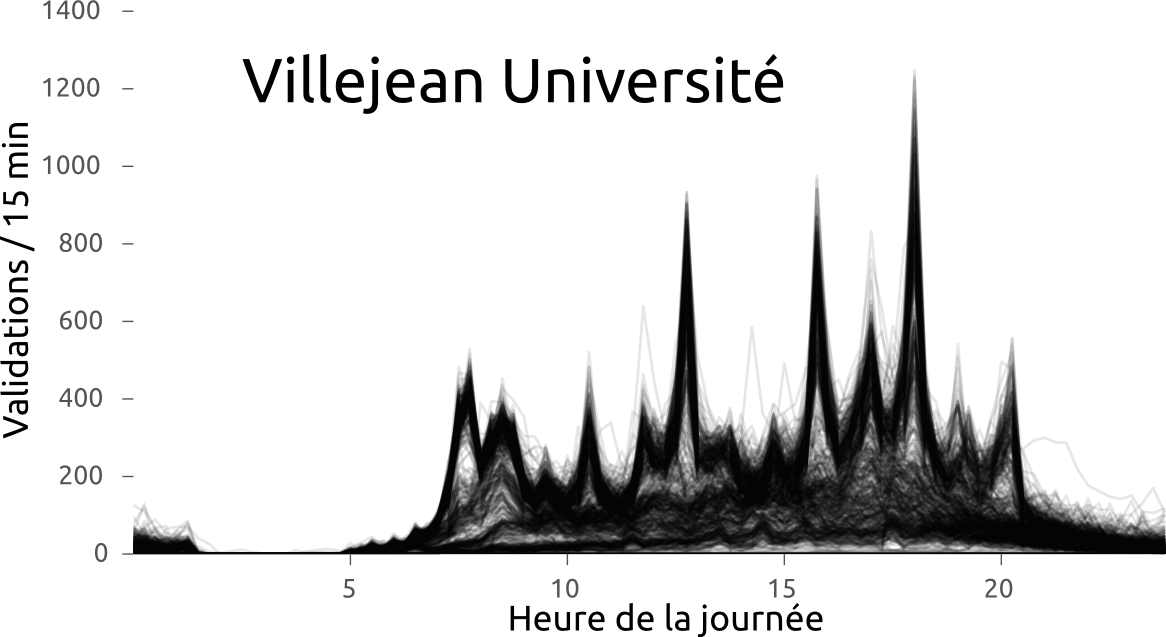### Between day variations clearly visible (CAH)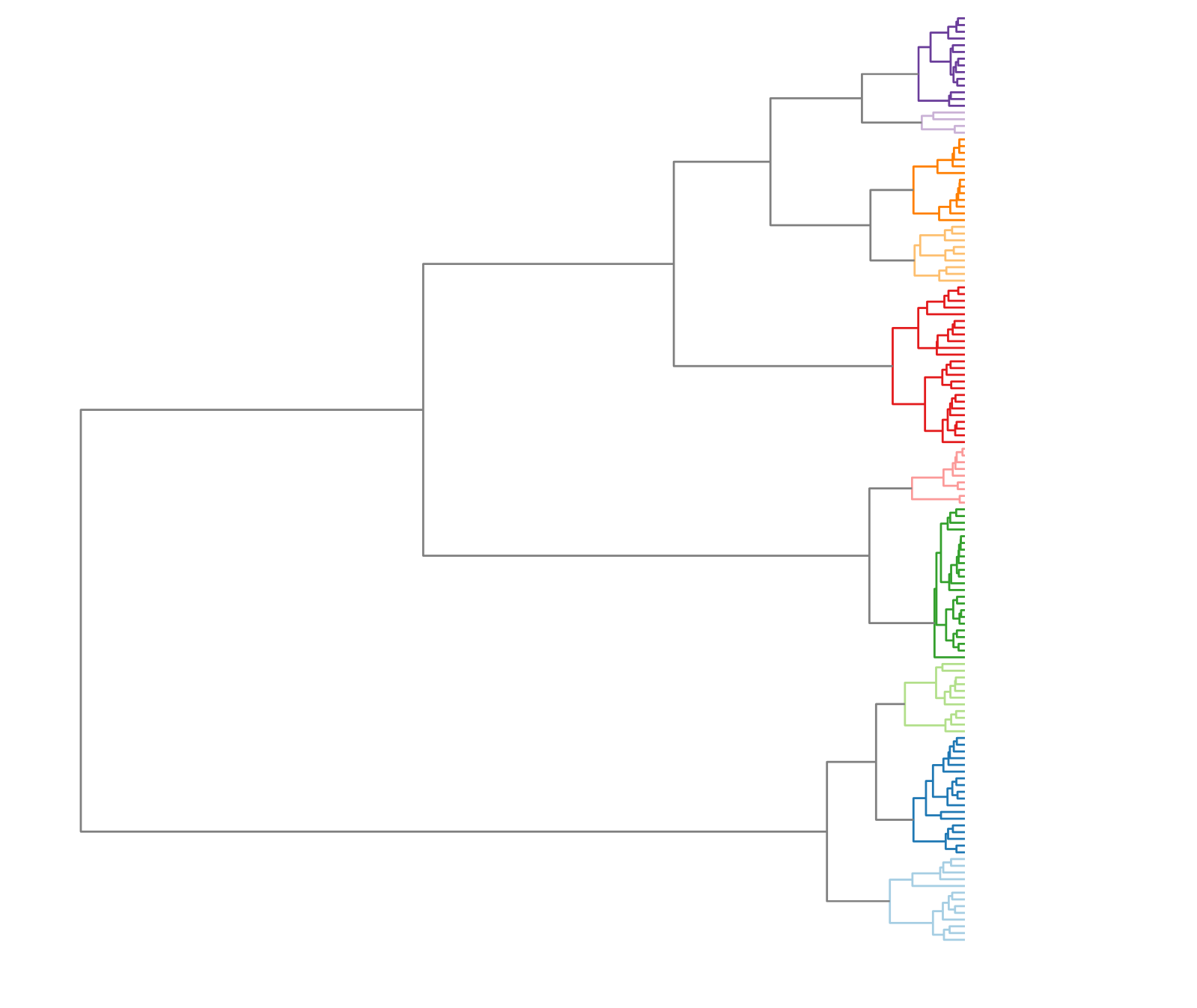### Between day variations clearly visible (CAH)### Between day variations clearly visible (CAH)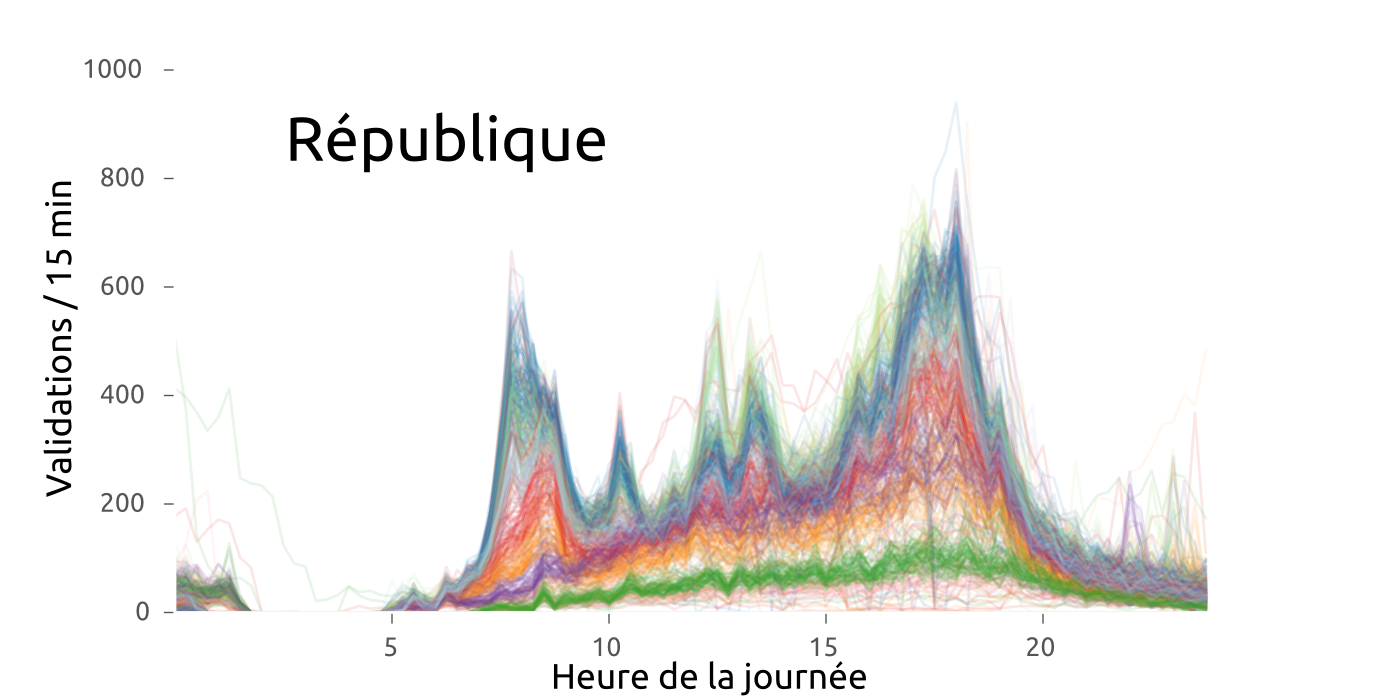### Between day variations clearly visible (CAH)### Between day variations clearly visible (CAH)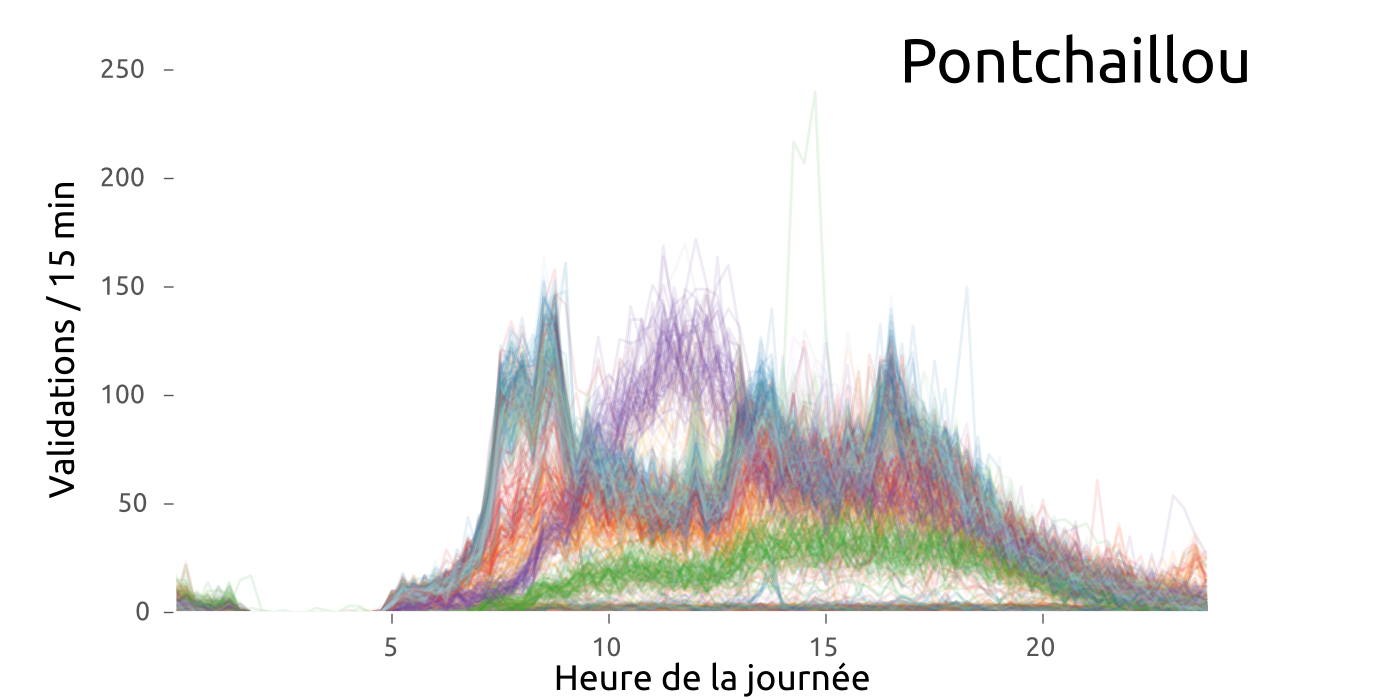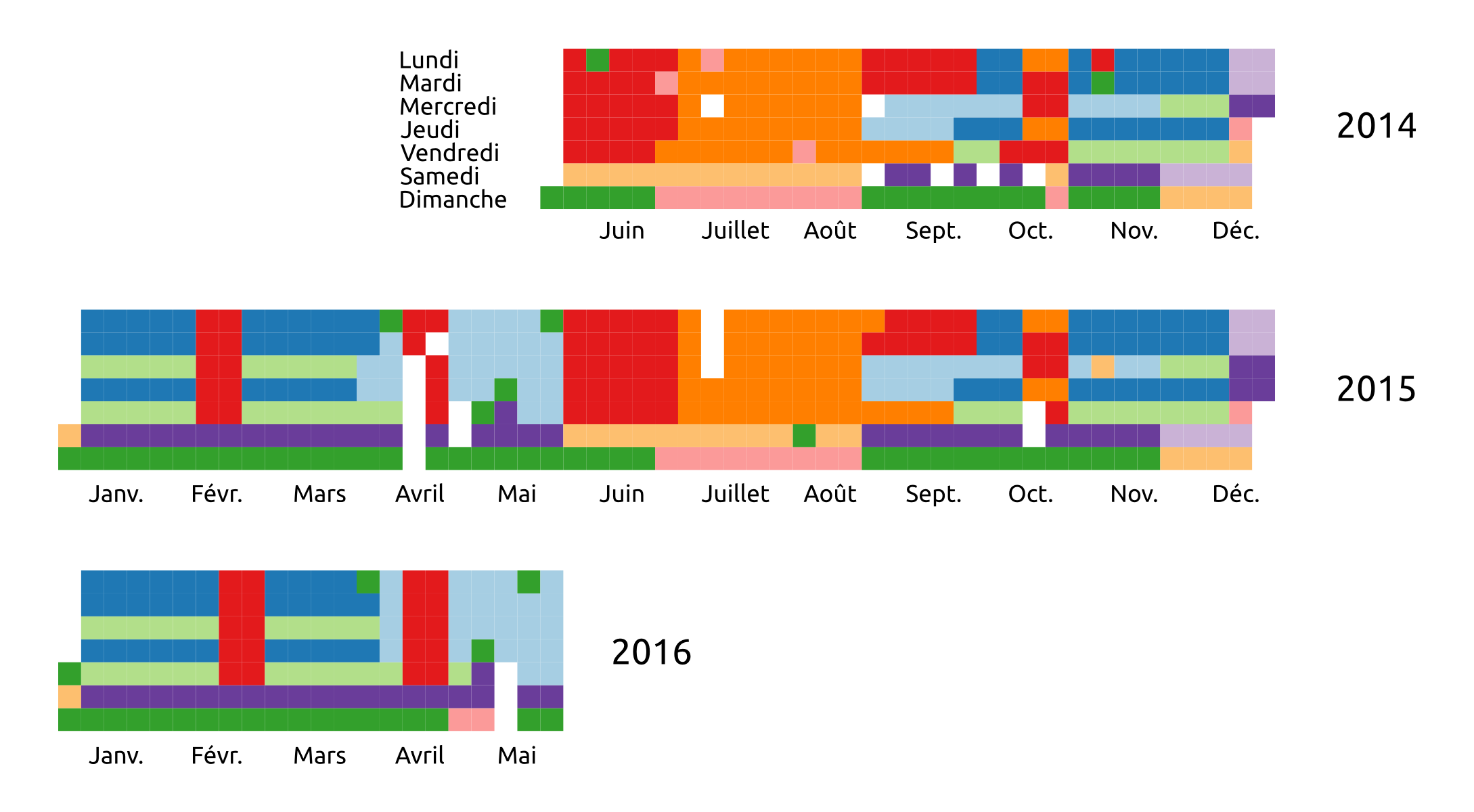#### Which is mainly explained by calendar effects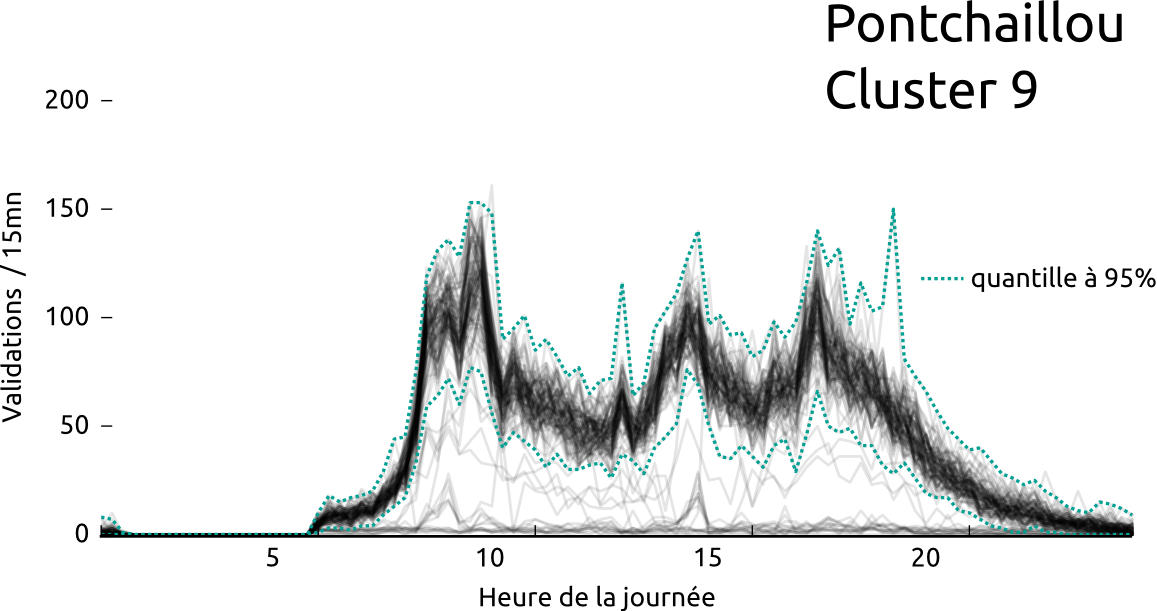#### Which can be used to detects outliers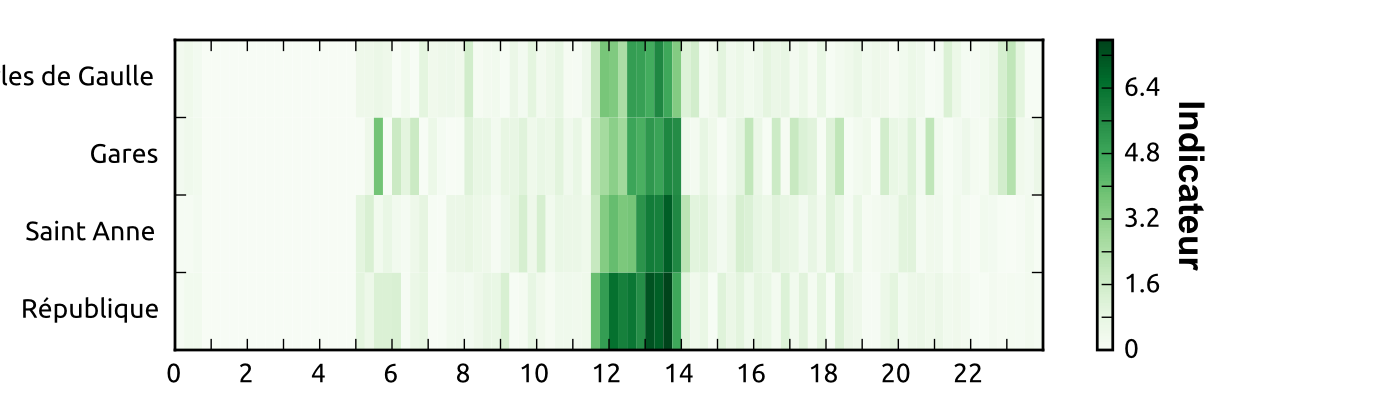#### Which can be used to detects outliers

#Rennes #metro #Star des chaises jetées sur la ligne aérienne de métro à Villejean. Dégâts importants. Trafic interrompu pendant 2h?

— Samuel Nohra (@SamuelNohra) 29 mars 2016#### Or perform mid-term predictions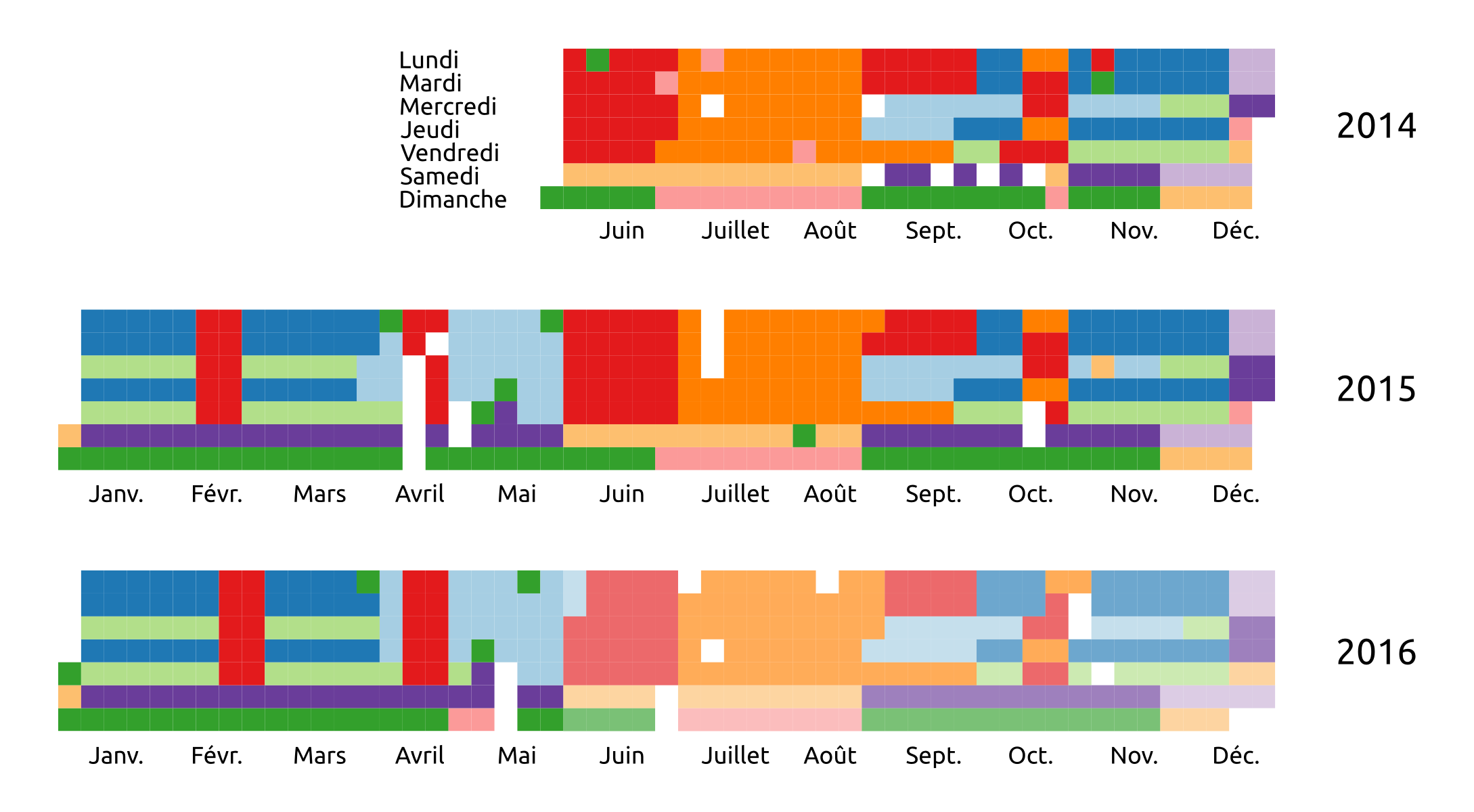# data enrichment

### Enable the reconstruction of a significant portion of the destinations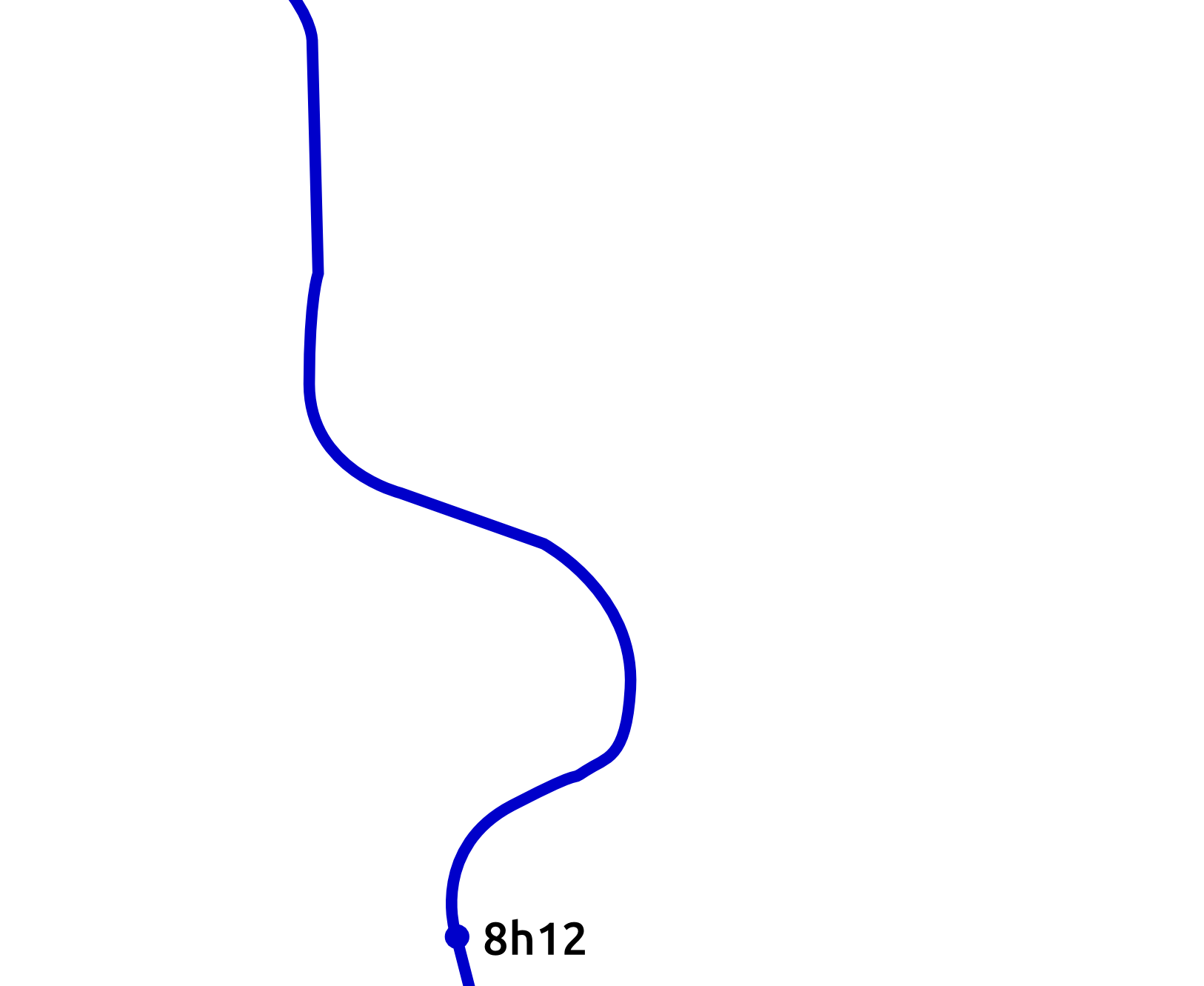### Enable the reconstruction of a significant portion of the destinations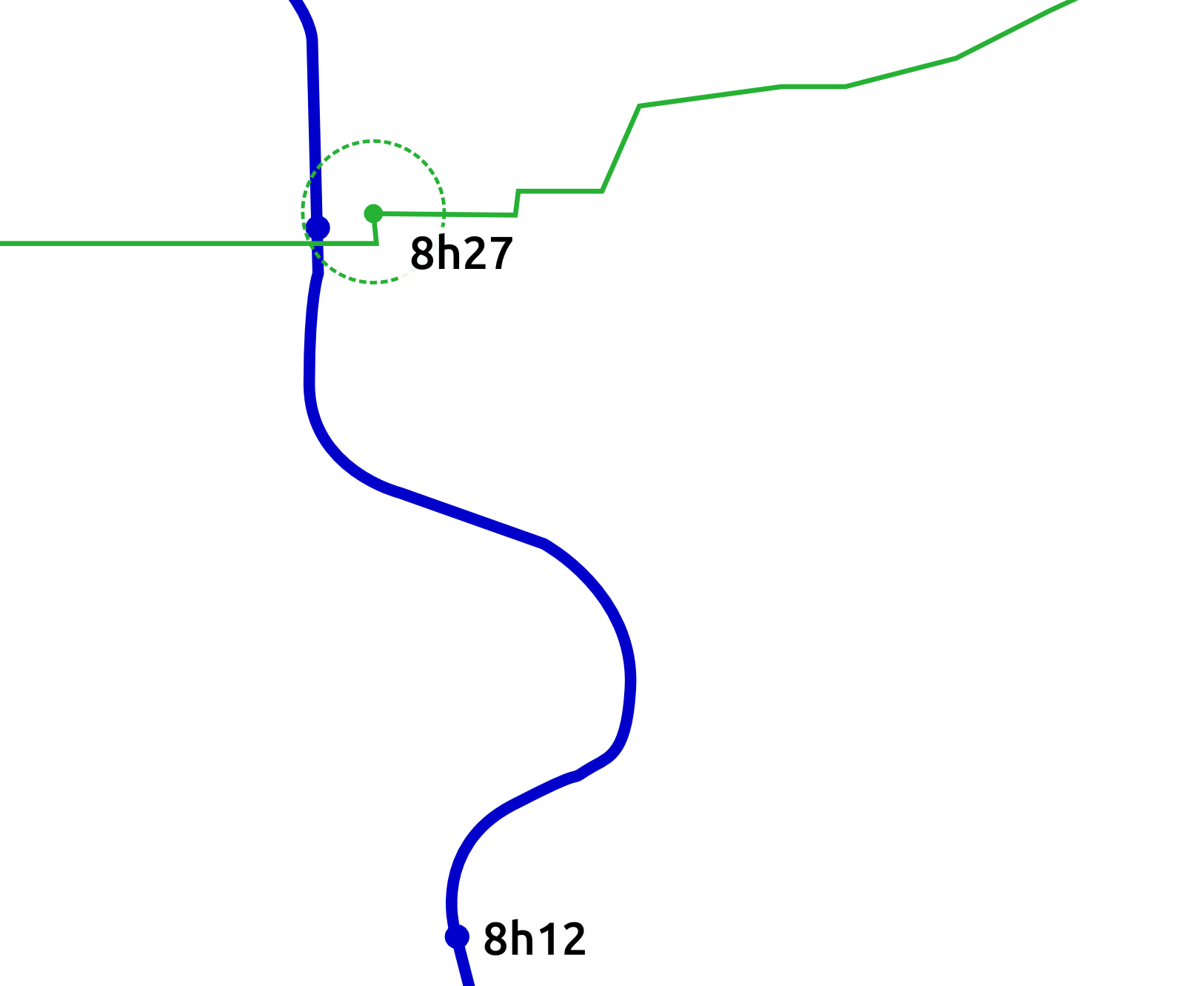### Enable the reconstruction of a significant portion of the destinations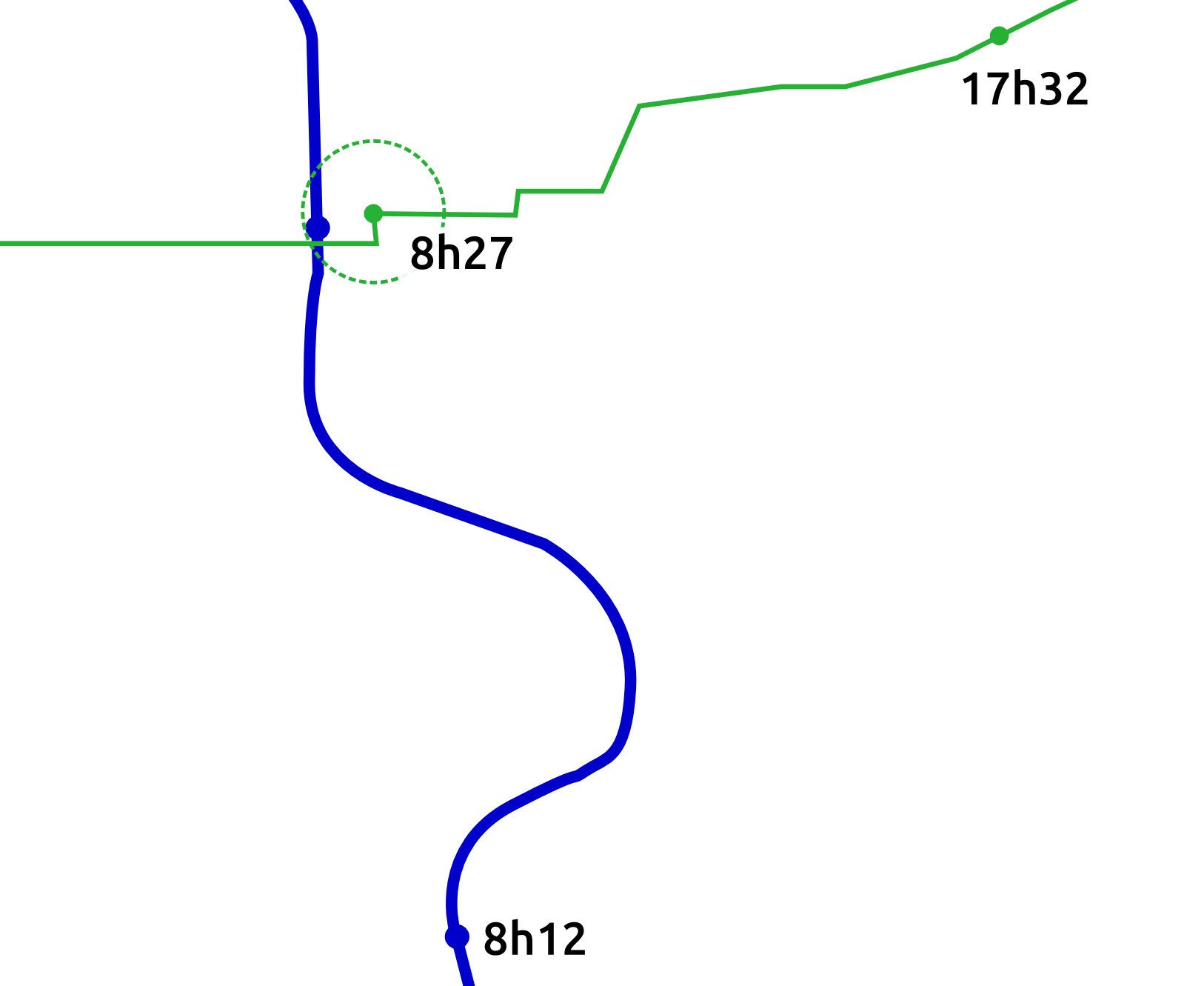### Enable the reconstruction of a significant portion of the destinations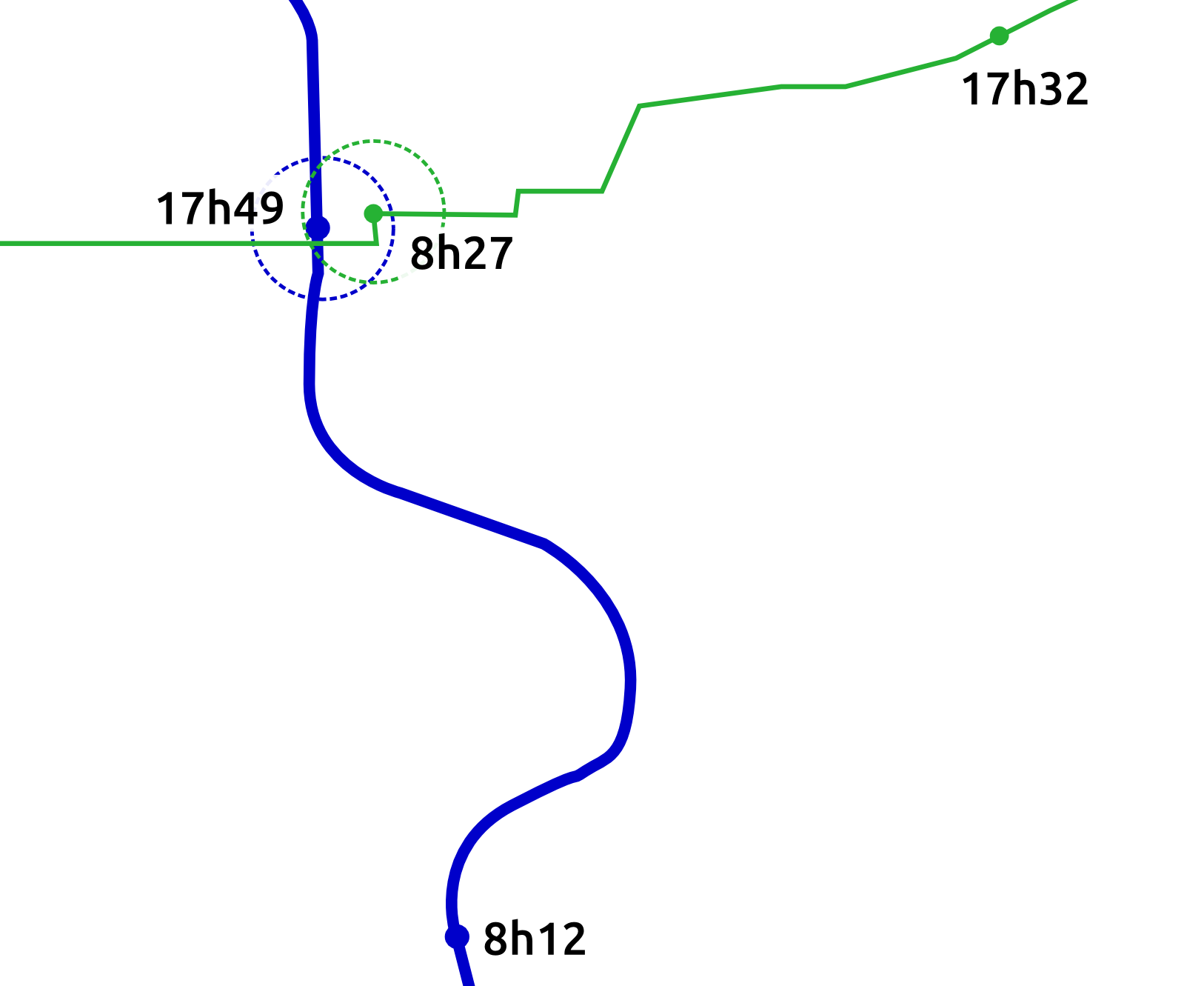### Enable the reconstruction of a significant portion of the destinations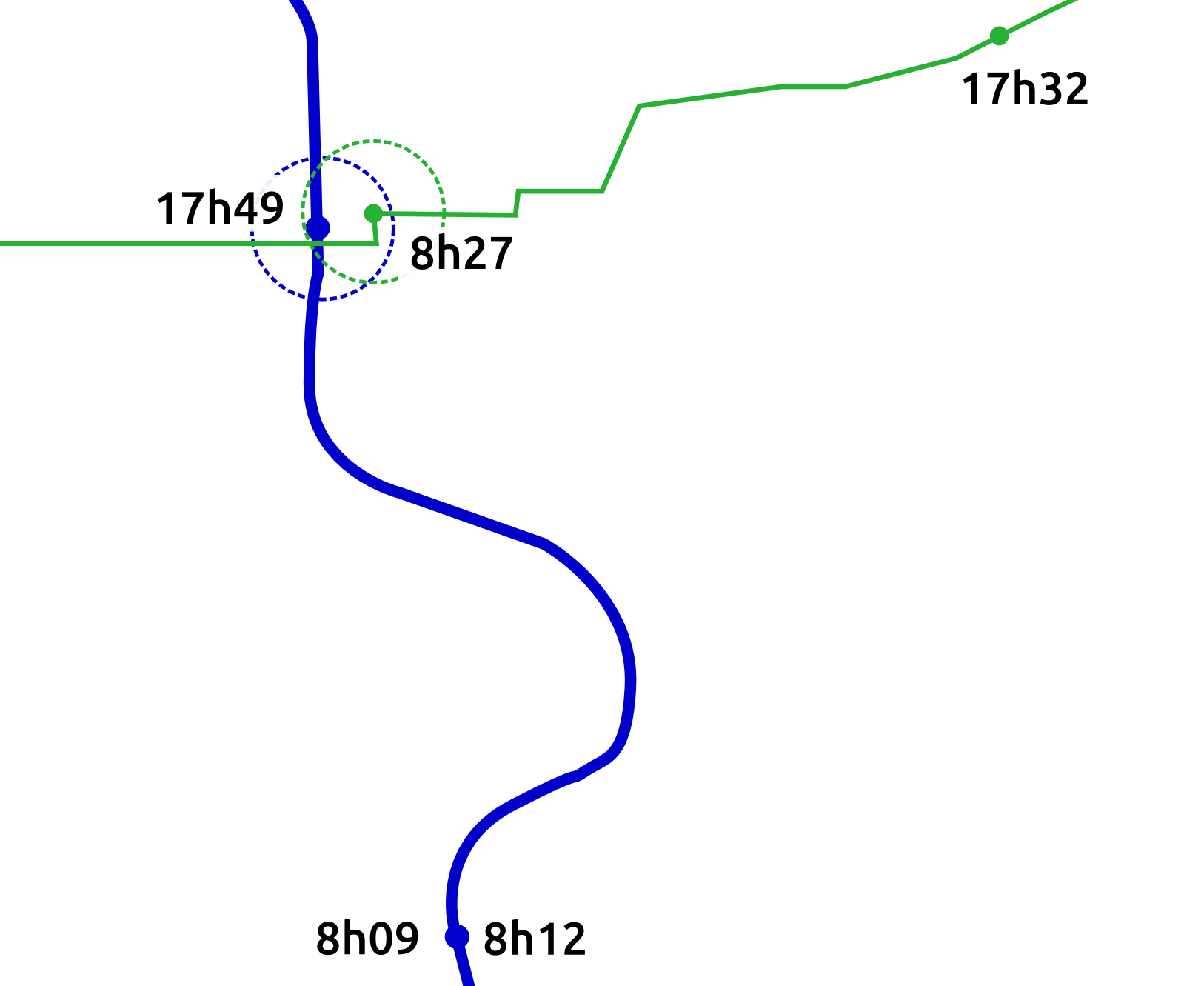# Data enrichment

• Use of next departure station to infer destination
• Temporal and spatial threshold
• Transfers detection
• User's ids must be kept 48h at minimum

### 72% of reconstructed destinations

→ Aggregation and analysis per Oirigines/Destinations
→ Multimodal exchange hub analysis (C. Richer)
→ Dynamic OD matrices or Line graph of load

# for user centric analysis

#### Objectives

• Study temporal regularity of user behaviors
• Understand how the global demand is decomposed
• Better understand users

#### Methodology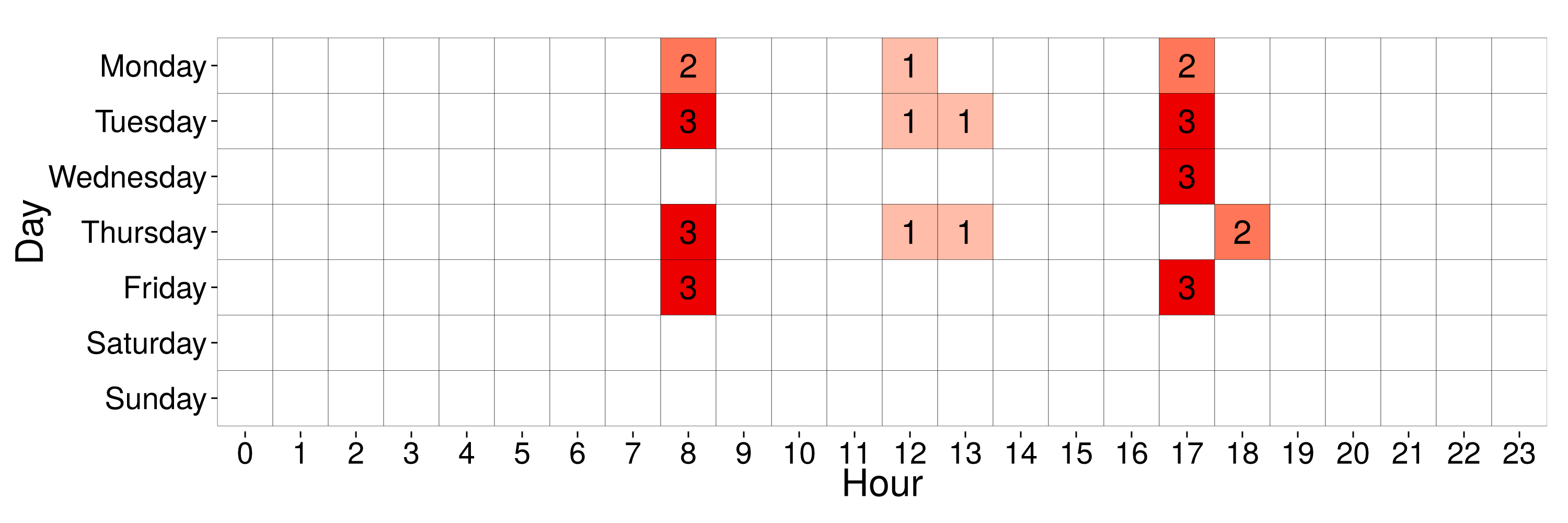• Build user profiles

#### Objectives

• Study temporal regularity of user behaviors
• Understand how the global demand is decomposed
• Better understand users

#### Methodology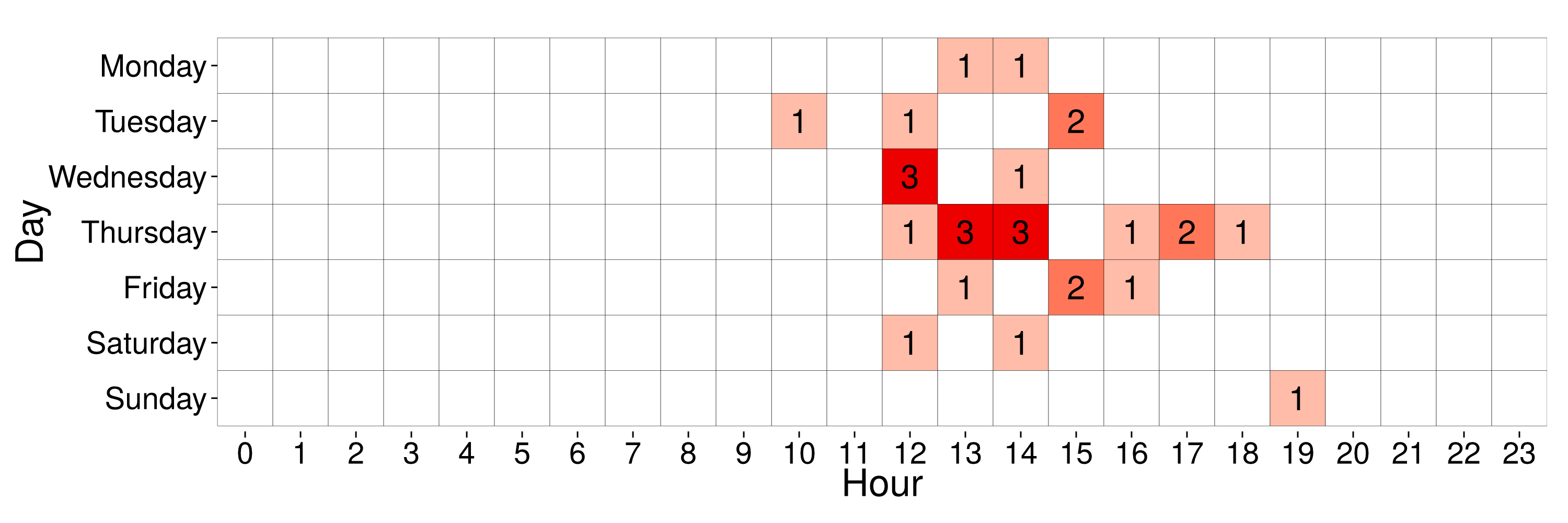• Build user profiles

# Commuter patterns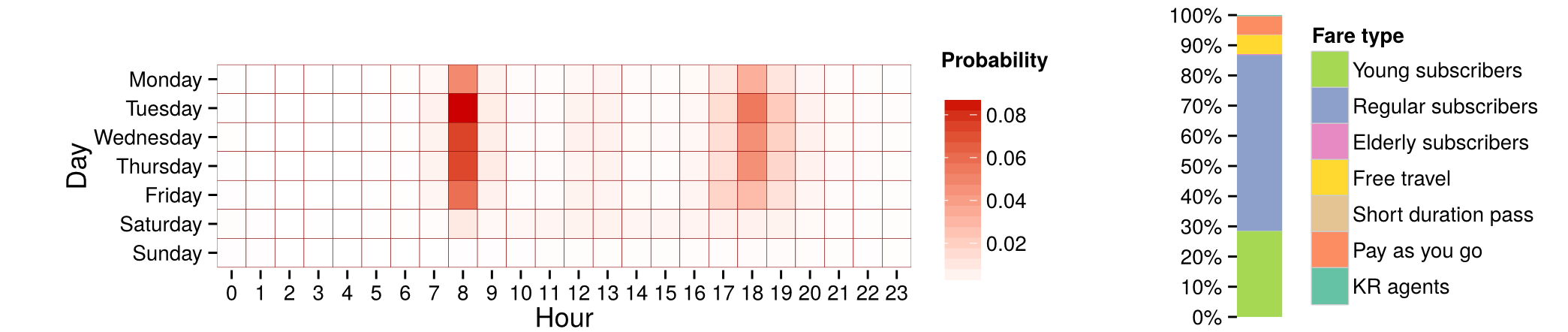Mean profile of a cluster with 4.55% of users

# Commuter patterns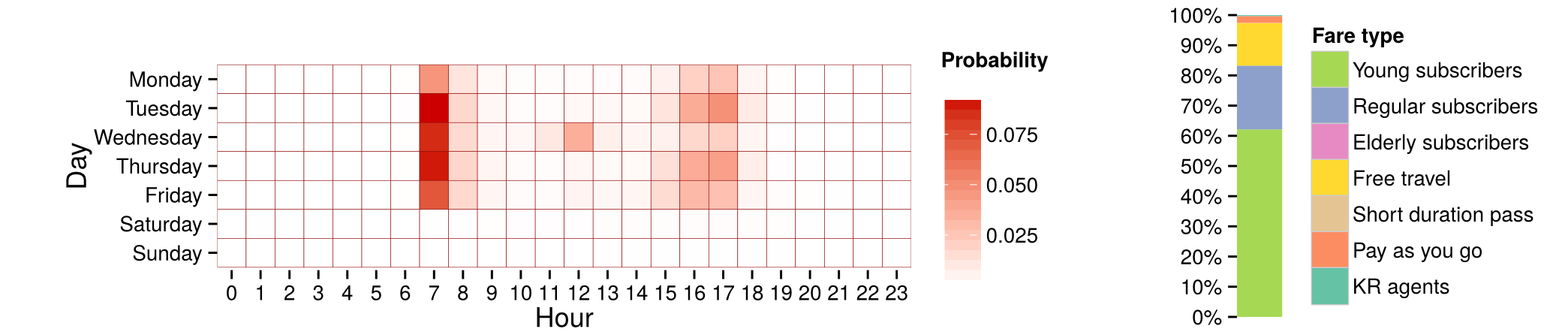Mean profile of a cluster with 12.54% of users

# Commuter patterns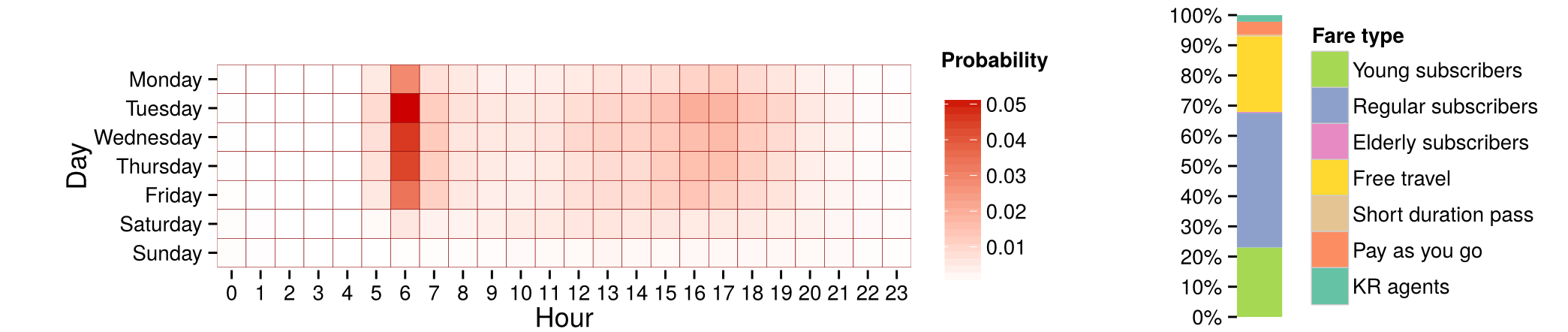Mean profile of a cluster with 3.6% of users

# But other forms emerge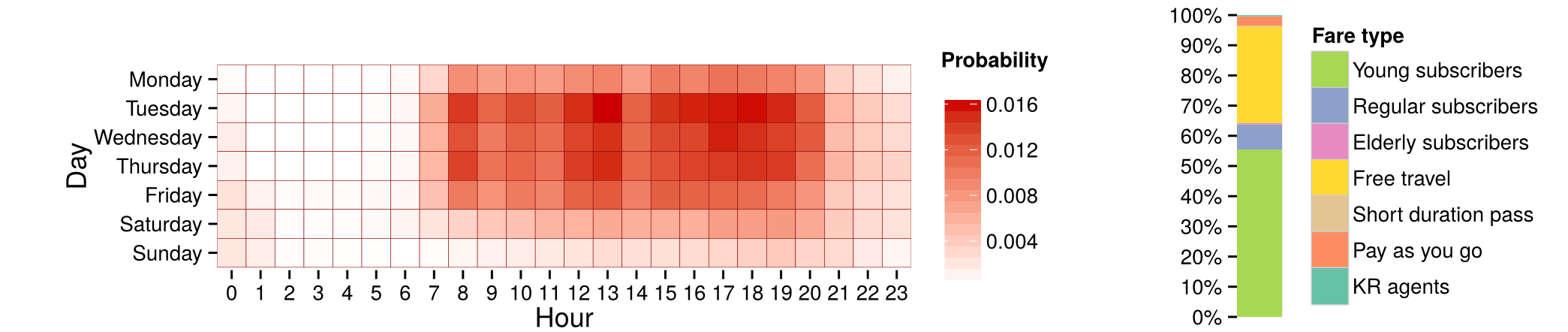Mean profile of a cluster with 15.13% of users

# But other forms emerge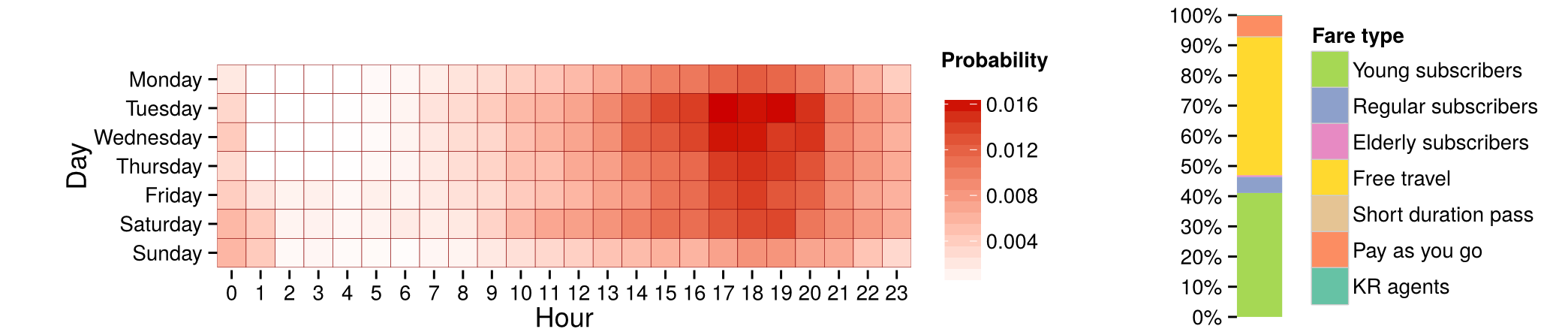Mean profile of a cluster with 6.44% of users

# But other forms emerge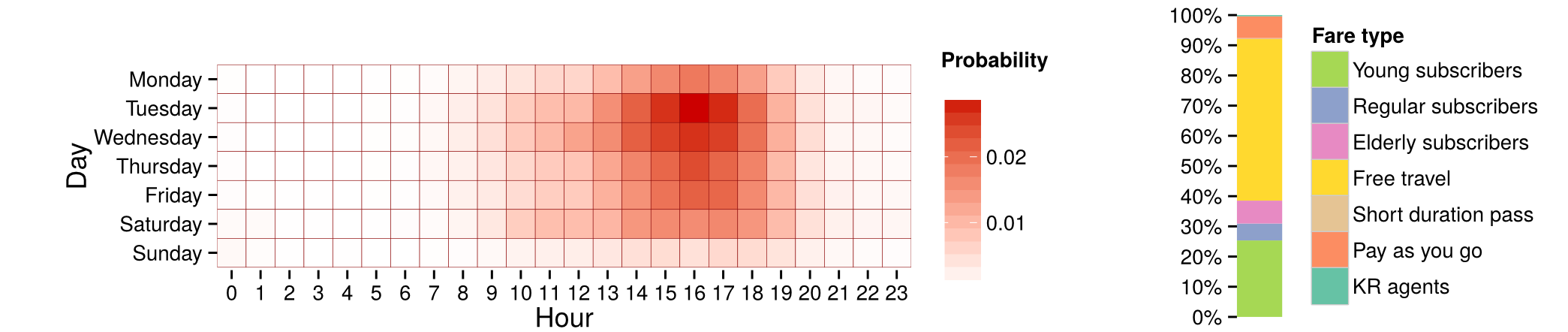Mean profile of a cluster with 8.64% of users

## Methodology

Using a continuous time description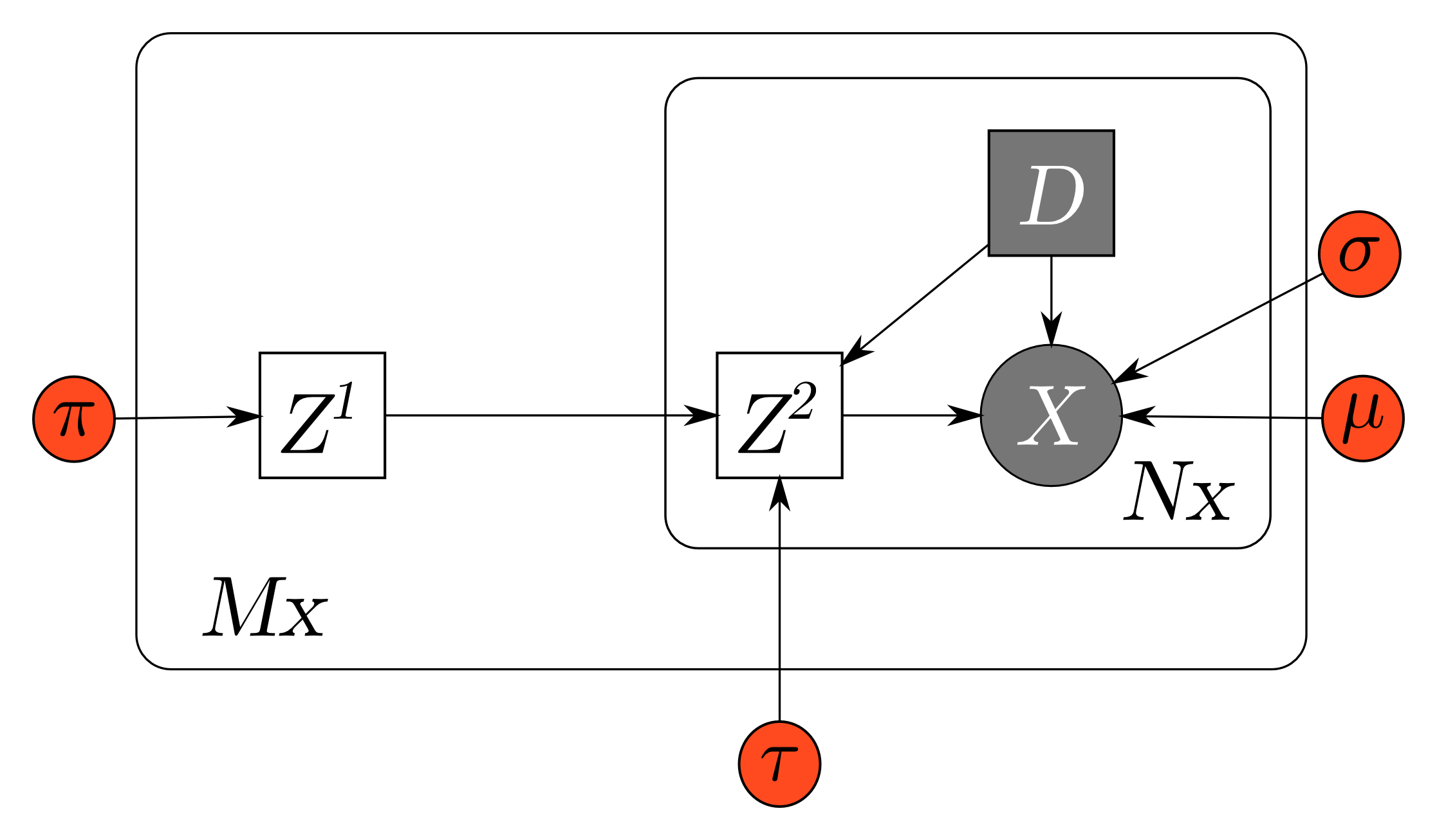Generative model for continuous time user clustering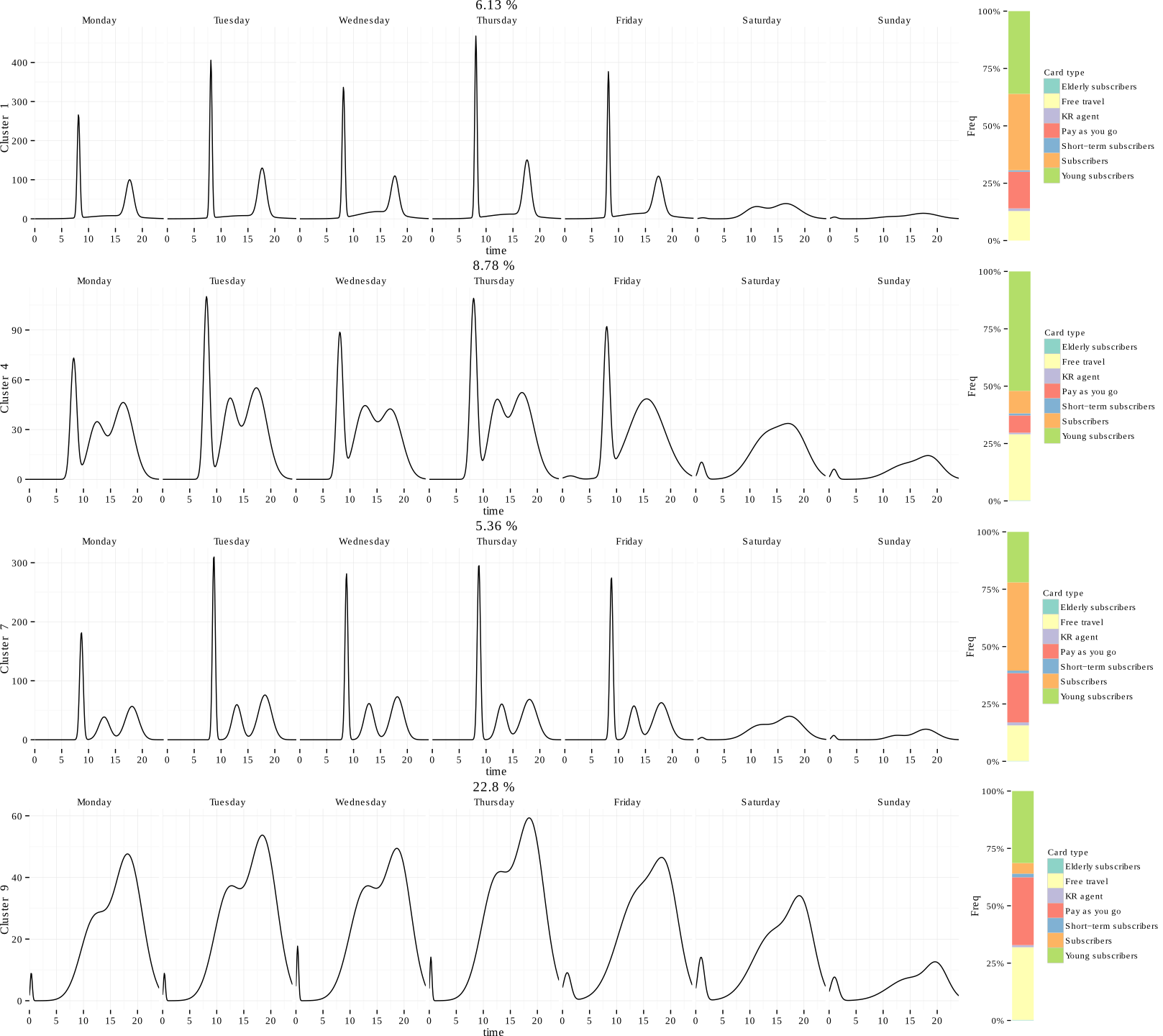# Some comments on the results

• Sub population quite different
• A quantitative view of user profile proportions
• Better understanding the user needs

#### Conclusion

• Smart card data are rich and enable a analysis of spatial and temporal variability of the demand
• Exploratory analysis to highlight these variations
• User's id are interesting to reconstruct destination and

#### Current works

• Model selection and bayesian regularisation for discrete latent variable models
$\Rightarrow$ Greedy optimization of Integrated Likelihood criterion
• Mid-term, short-term prediction of in-flow a
• Anomaly detection and characterization
• Work on larger networks
• Network of users interactions

# Bibliographie

El Mahrsi, M., Briand, A. S., Côme, E., & Oukhellou, L. "Utilité des données billettiques pour l’analyse des mobilités urbaines: le cas rennais" Données urbaines, Economica, 2015, 11p.
El Mahrsi, M., Côme, E., Oukhellou, L. & Verleysen, M. "Clustering Smart Card Data for Urban Mobility Analysis" Ieee Transactions on Intelligent Transportation Suystems ( Volume: PP, Issue: 99 ), pp 1 – 17, 2016.
Briand, A. S., Côme, E., El Mahrsi, M., & Oukhellou, L. "A mixture model clustering approach for temporal passenger pattern characterization in public transport", International Journal of Data Science and Analytics, Avril 2016, Volume 1, issue 1, pp 37-50.
Briand, A. S., Côme, E., El Mahrsi, M., & Oukhellou, L. "Classification á base de Modèle de mélange pour l’identification de profils temporels types d’usagers de transport public" AAFD & SFC’16 : Conférence Internationale Francophone sur la Science des Données, Marrakech, 2016.
Toqué, F., Côme, E., El Mahrsi, M., Oukhellou, L. "Forecasting Dynamic Public Transport Origin-Destination Matrices with Long-Short Term Memory Recurrent Neural Networks". In Proceedings of IEEE 19th International Conference on Intelligent Transportation Systems , Rio de Janeiro , BRESIL 2016.

# Bibliographie

#### Work in progress :

Briand, A. S., Côme, E., Trépanier, M., & Oukhellou, L. "Analysing year-to-year changes in public transport passenger behaviour using smart card data" Transportation Research Part C : Special Issue on Smartcard data (article accepté en cours de publication)
Briand, A. S., Côme, E., & Oukhellou, L. "Anomalie detection and characterization in Smart-Card data" (article en cours de finalisation)